Скачать презентацию Lecture 7: Voronoi Diagrams Presented by Allen

voronoi2d.ppt

• Размер: 483.0 Кб
• Автор: Максим Гончаренко
• Количество слайдов: 79

## Описание презентации Lecture 7: Voronoi Diagrams Presented by Allen по слайдам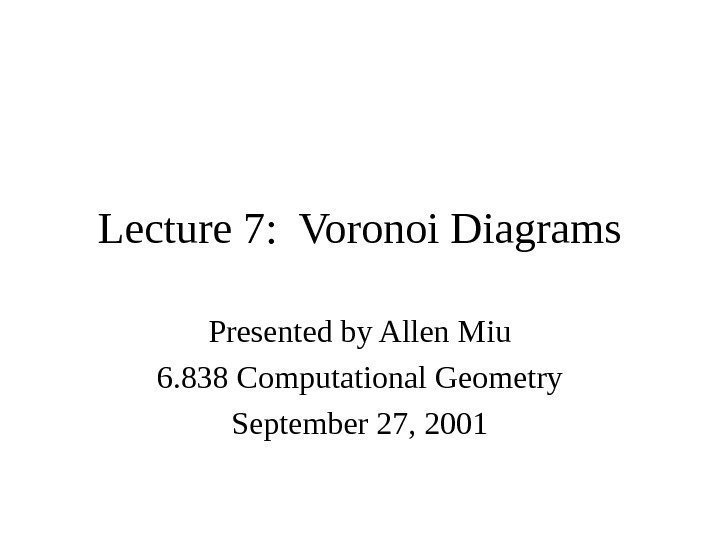Lecture 7: Voronoi Diagrams Presented by Allen Miu 6. 838 Computational Geometry September 27,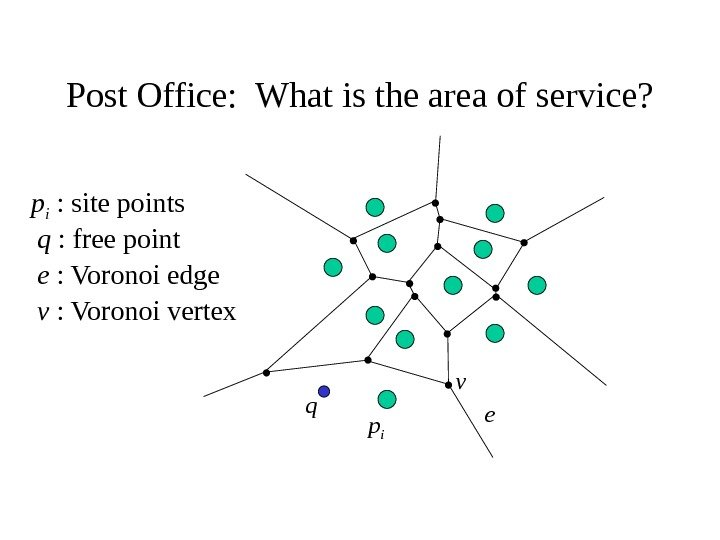Post Office: What is the area of service? qq : free point ee : Voronoi edge vv : Voronoi vertex p ip i : site points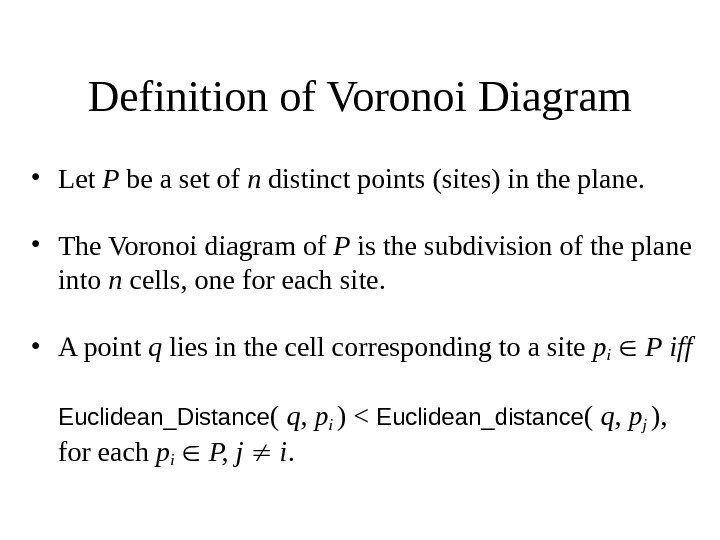Definition of Voronoi Diagram • Let P be a set of n distinct points (sites) in the plane. • The Voronoi diagram of P is the subdivision of the plane into n cells, one for each site. • A point q lies in the cell corresponding to a site pi P iff Euclidean_Distance ( q , p i ) < Euclidean_distance ( q , pj ), for each p i P, j i.Demo http: //www. diku. dk/students/duff/Fortune/ http: //www. msi. umn. edu/~schaudt/voronoi/ voronoi. html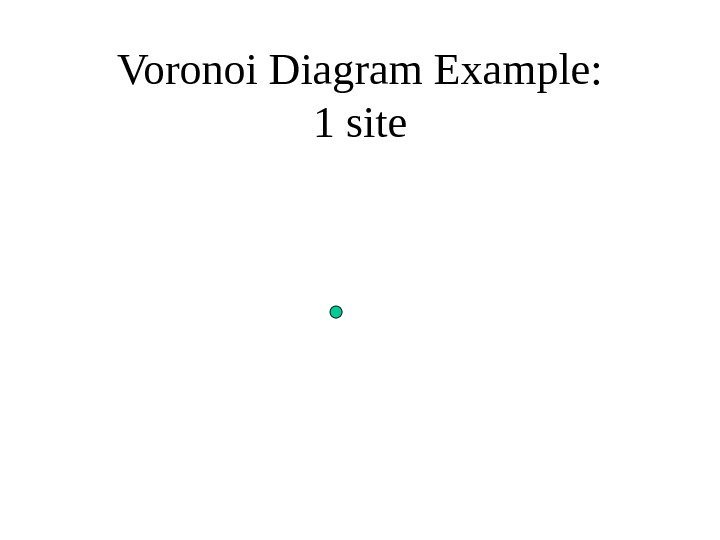Voronoi Diagram Example: 1 site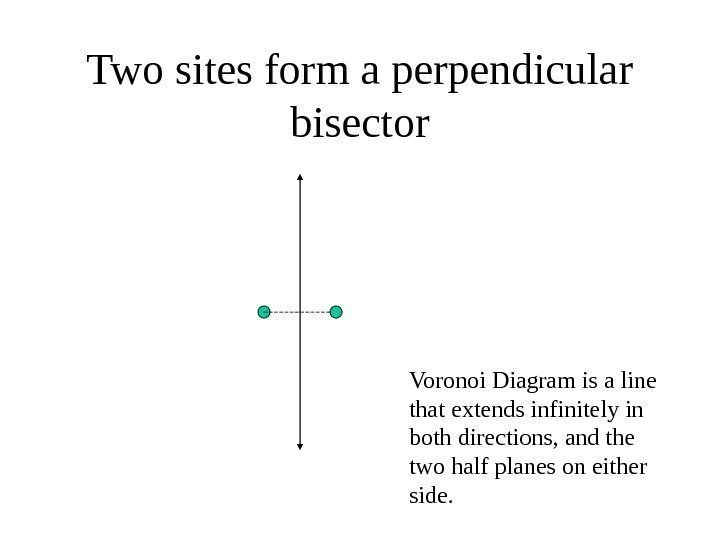Two sites form a perpendicular bisector Voronoi Diagram is a line that extends infinitely in both directions, and the two half planes on either side.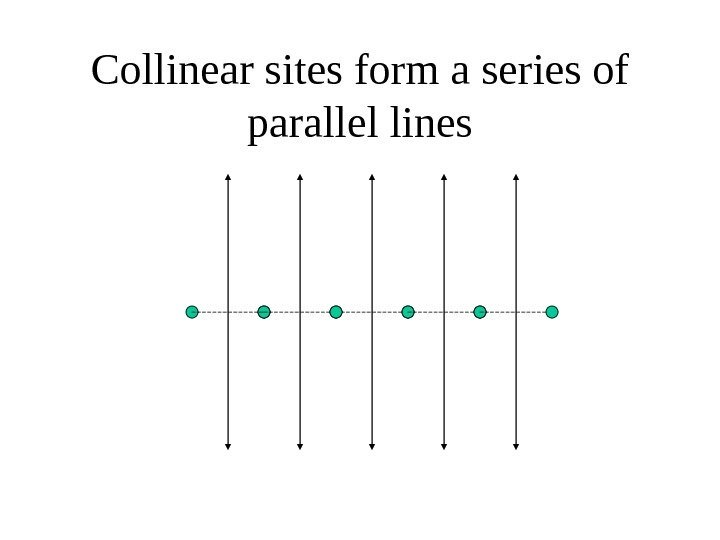Collinear sites form a series of parallel lines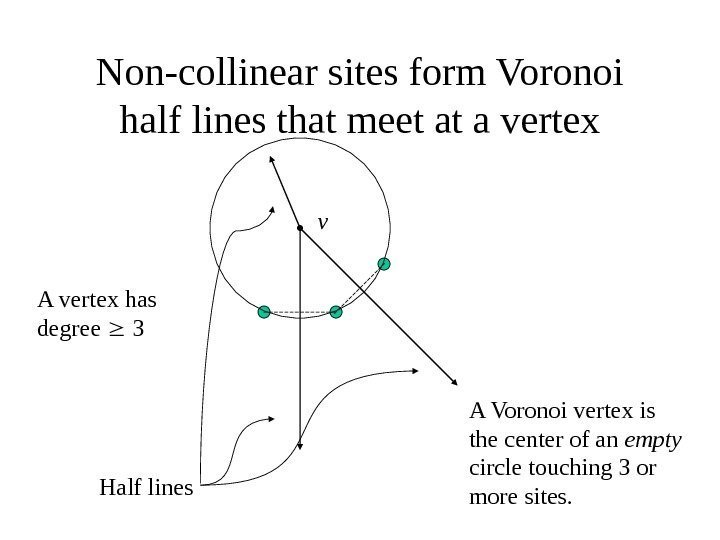Non-collinear sites form Voronoi half lines that meet at a vertex A Voronoi vertex is the center of an empty circle touching 3 or more sites. v Half lines. A vertex has degree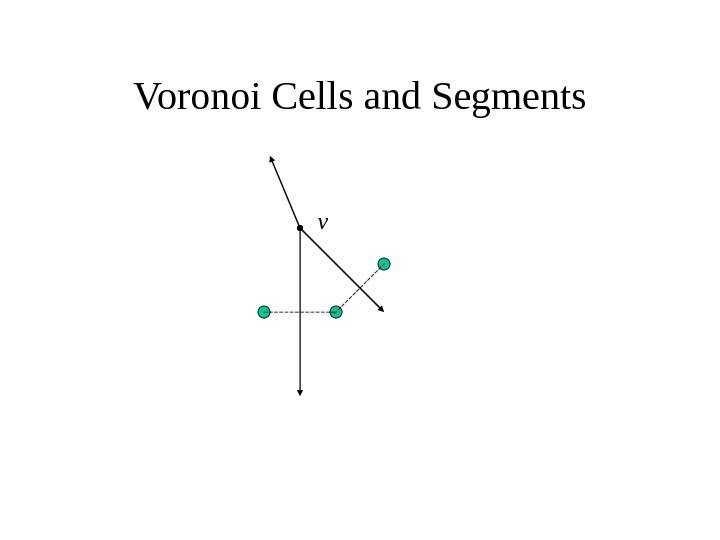Voronoi Cells and Segments v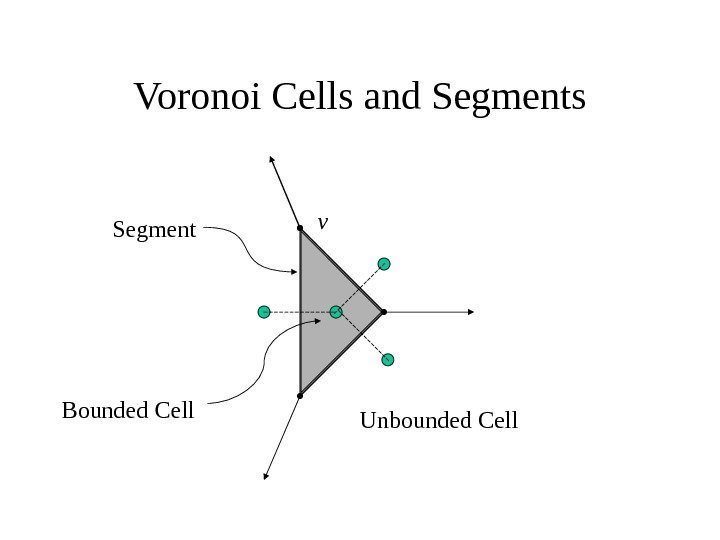Voronoi Cells and Segments v Unbounded Cell. Bounded Cell Segment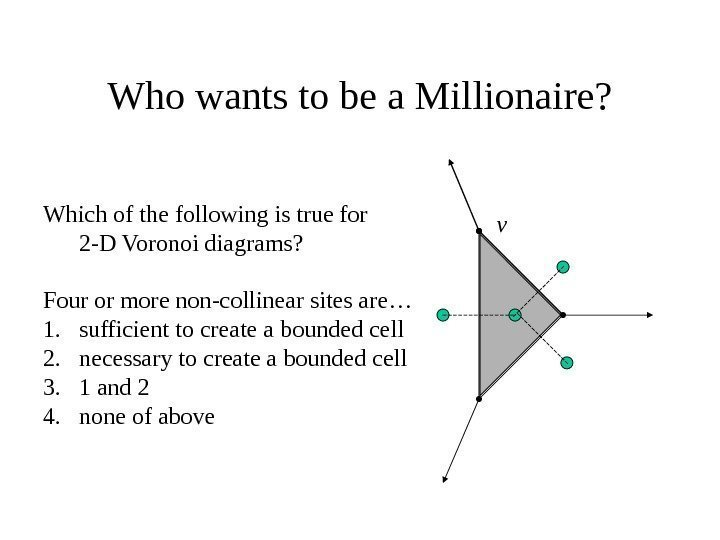Who wants to be a Millionaire? v. Which of the following is true for 2 -D Voronoi diagrams? Four or more non-collinear sites are… 1. sufficient to create a bounded cell 2. necessary to create a bounded cell 3. 1 and 2 4. none of above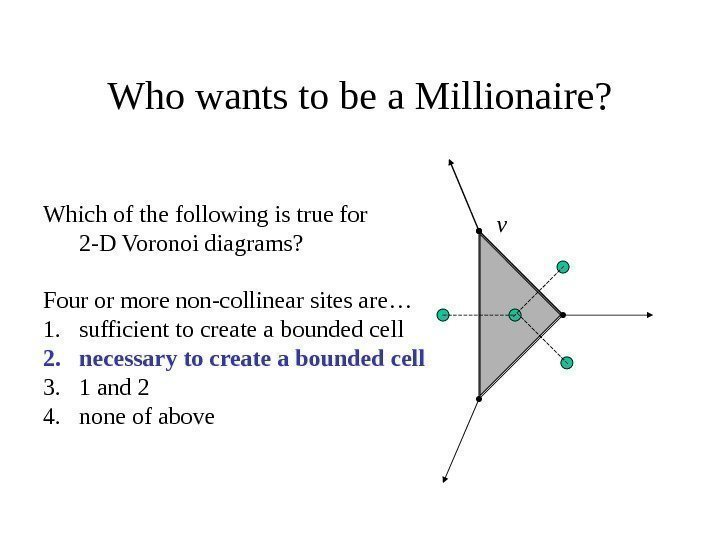Who wants to be a Millionaire? v. Which of the following is true for 2 -D Voronoi diagrams? Four or more non-collinear sites are… 1. sufficient to create a bounded cell 2. necessary to create a bounded cell 3. 1 and 2 4. none of above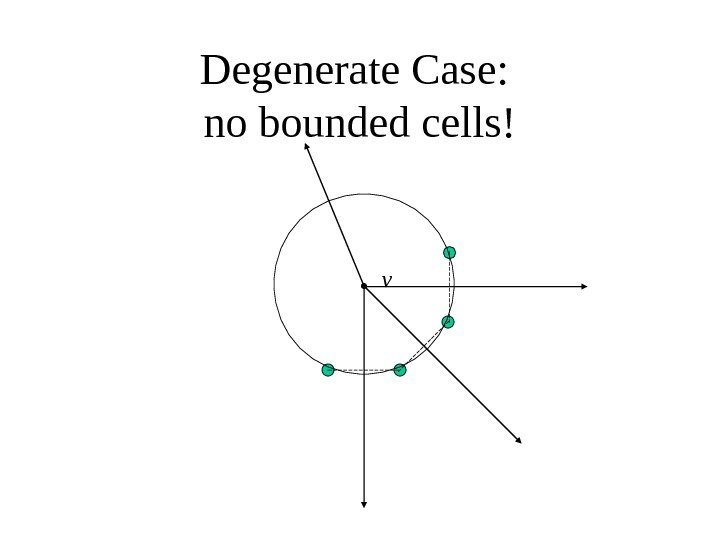Degenerate Case: no bounded cells! v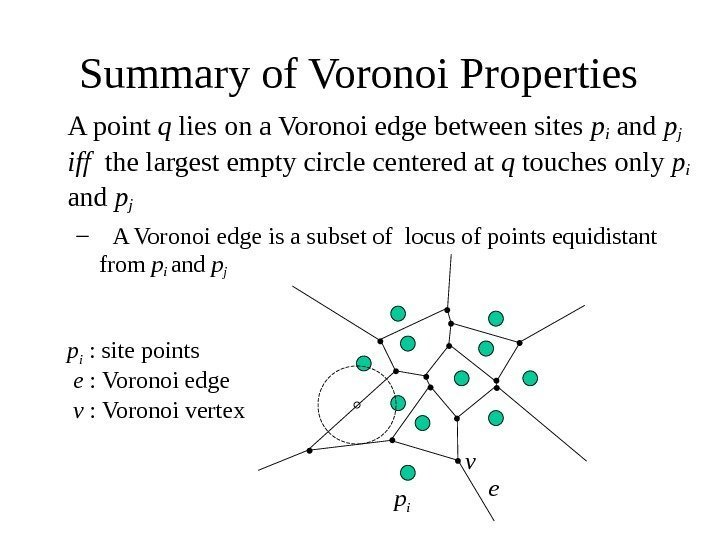Summary of Voronoi Properties A point q lies on a Voronoi edge between sites pi and pj iff the largest empty circle centered at q touches only p i and p j – A Voronoi edge is a subset of locus of points equidistant from p i and pj ee : Voronoi edge vv : Voronoi vertex p ip i : site points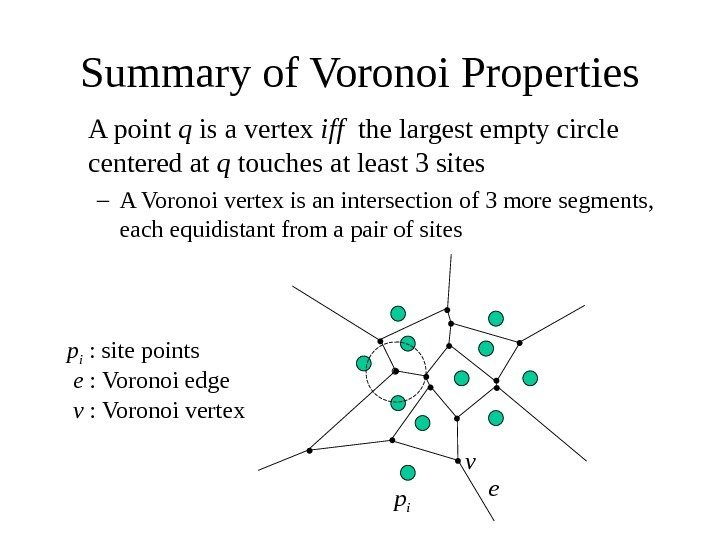Summary of Voronoi Properties A point q is a vertex iff the largest empty circle centered at q touches at least 3 sites – A Voronoi vertex is an intersection of 3 more segments, each equidistant from a pair of sites ee : Voronoi edge vv : Voronoi vertex p ip i : site points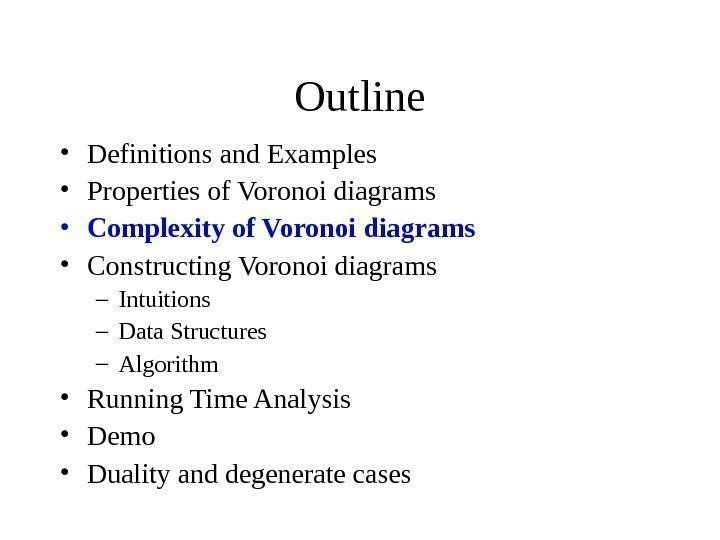Outline • Definitions and Examples • Properties of Voronoi diagrams • Complexity of Voronoi diagrams • Constructing Voronoi diagrams – Intuitions – Data Structures – Algorithm • Running Time Analysis • Demo • Duality and degenerate cases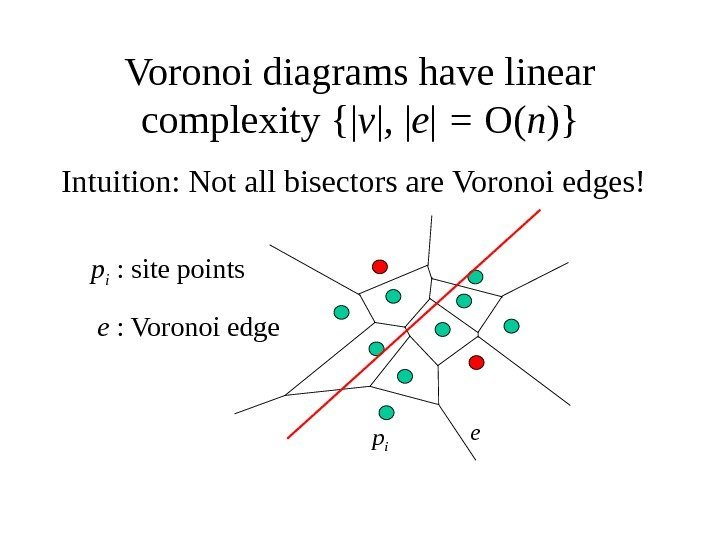Voronoi diagrams have linear complexity {| v | , | e | = O( n )} Intuition: Not all bisectors are Voronoi edges! ee : Voronoi edge p ip i : site points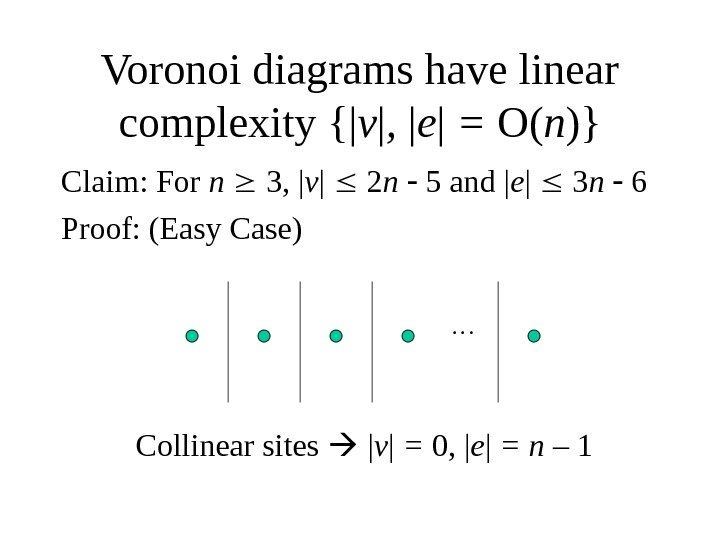Voronoi diagrams have linear complexity {| v | , | e | = O( n )} Claim: For n 3, | v | 2 n 5 and | e | 3 n 6 Proof: (Easy Case) … Collinear sites | v | = 0, | e | = n –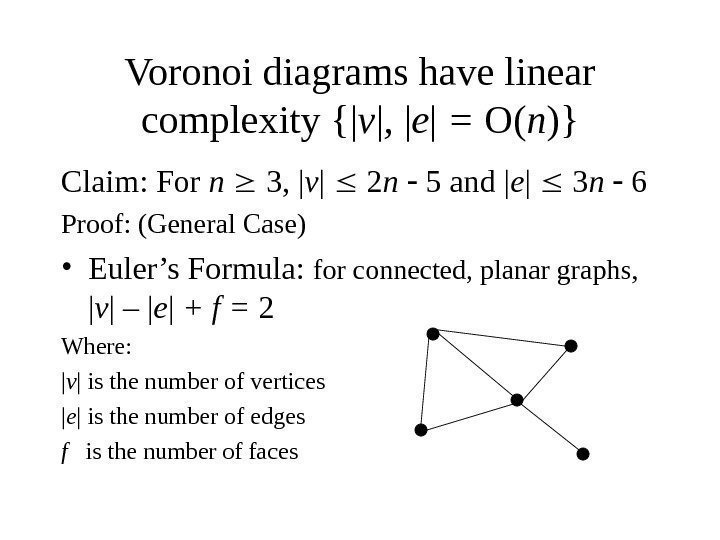Voronoi diagrams have linear complexity {| v | , | e | = O( n )} Claim: For n 3, | v | 2 n 5 and | e | 3 n 6 Proof: (General Case) • Euler’s Formula: for connected, planar graphs, | v | – | e | + f = 2 Where: | v | is the number of vertices | e | is the number of edges f is the number of faces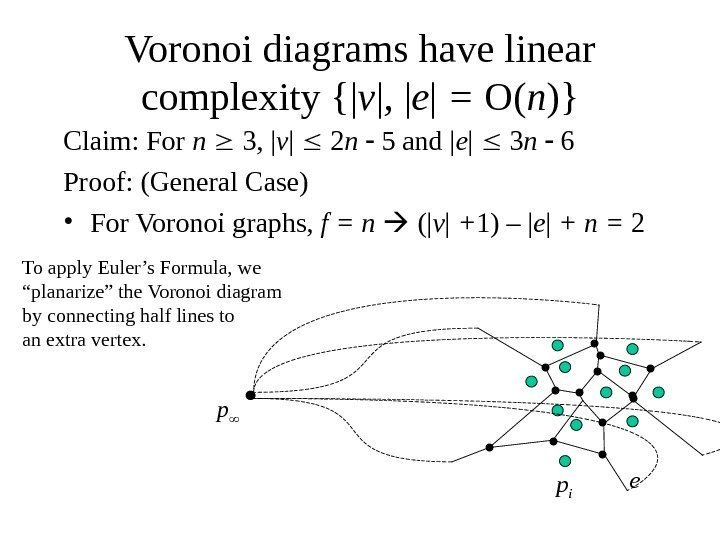Voronoi diagrams have linear complexity {| v | , | e | = O( n )} Claim: For n 3, | v | 2 n 5 and | e | 3 n 6 Proof: (General Case) • For Voronoi graphs, f = n (| v | + 1) – | e | + n = 2 e p ip To apply Euler’s Formula, we “ planarize” the Voronoi diagram by connecting half lines to an extra vertex.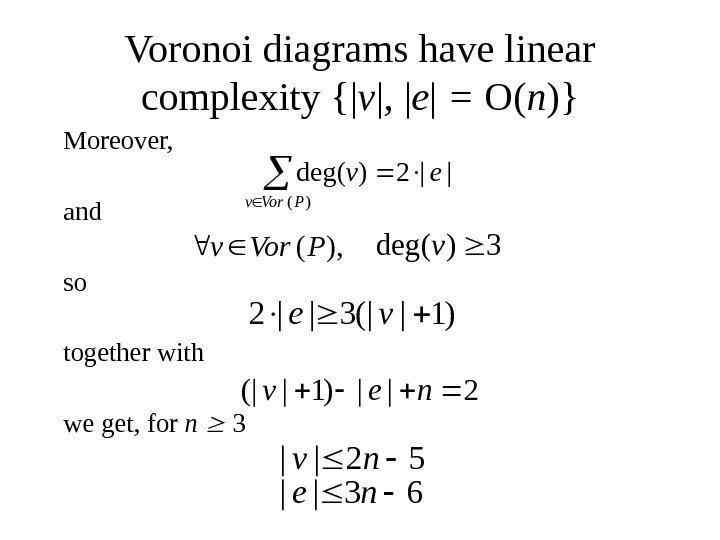Voronoi diagrams have linear complexity {| v | , | e | = O( n )} Moreover, and so together with we get, for n 3 ||2)deg( )( ev PVorv ), (PVorv 3)deg(v )1|(|3||2 ve 2||)1|(|nev 63|| 52|| ne nv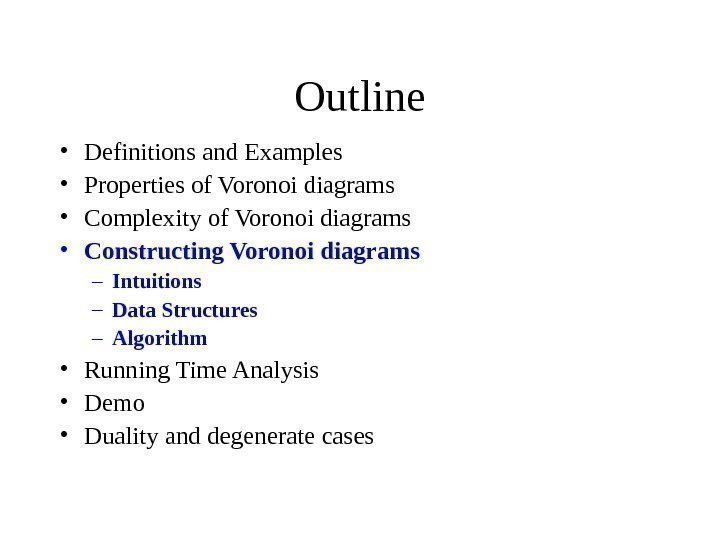Outline • Definitions and Examples • Properties of Voronoi diagrams • Complexity of Voronoi diagrams • Constructing Voronoi diagrams – Intuitions – Data Structures – Algorithm • Running Time Analysis • Demo • Duality and degenerate cases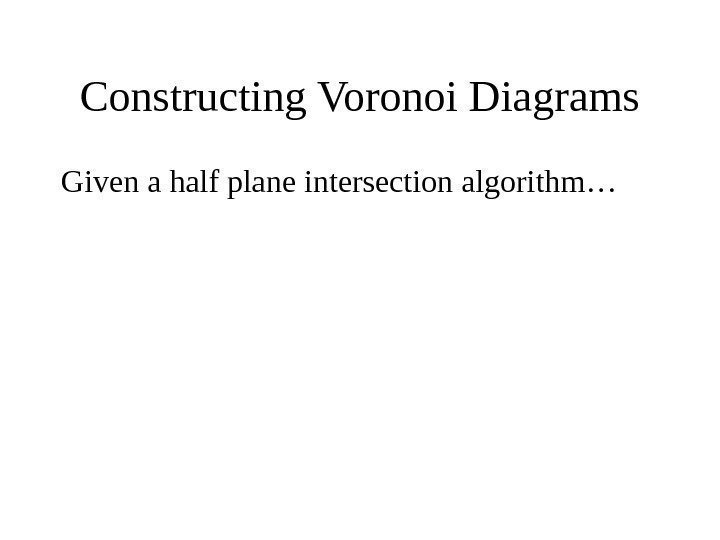Constructing Voronoi Diagrams Given a half plane intersection algorithm…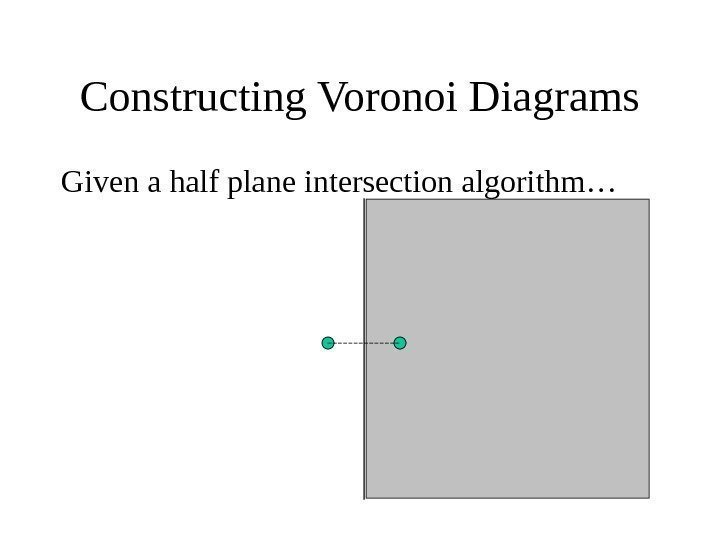Constructing Voronoi Diagrams Given a half plane intersection algorithm…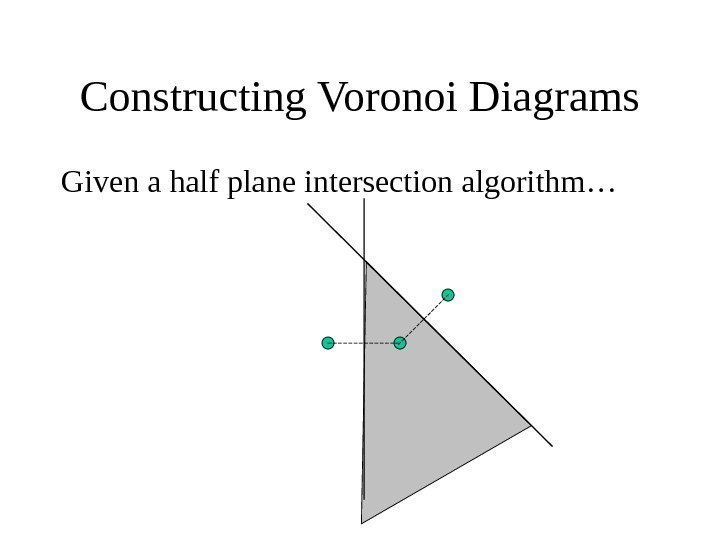Constructing Voronoi Diagrams Given a half plane intersection algorithm…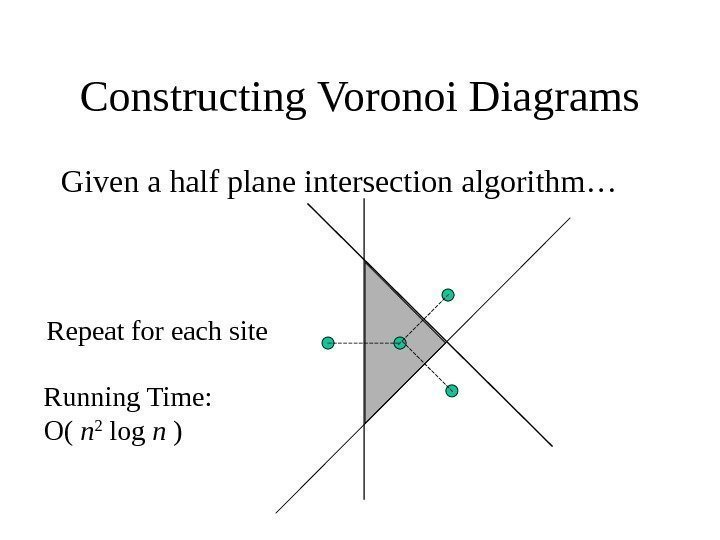Constructing Voronoi Diagrams Given a half plane intersection algorithm… Repeat for each site Running Time: O( n 2 log n )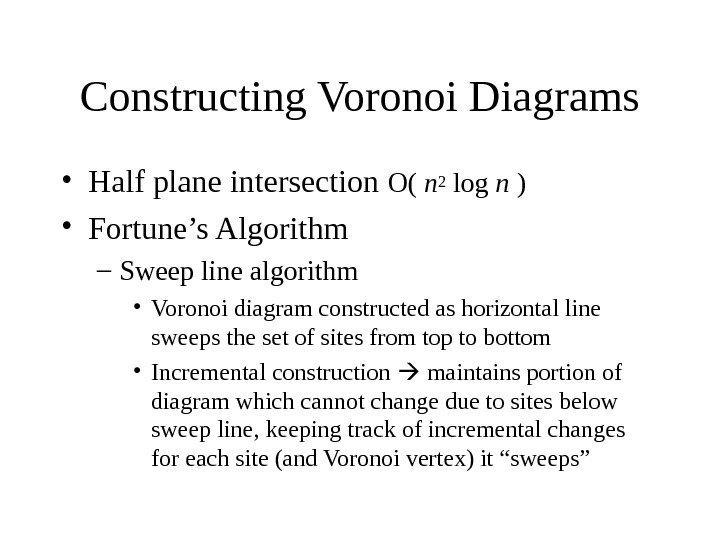Constructing Voronoi Diagrams • Half plane intersection O( n 2 log n ) • Fortune’s Algorithm – Sweep line algorithm • Voronoi diagram constructed as horizontal line sweeps the set of sites from top to bottom • Incremental construction maintains portion of diagram which cannot change due to sites below sweep line, keeping track of incremental changes for each site (and Voronoi vertex) it “sweeps”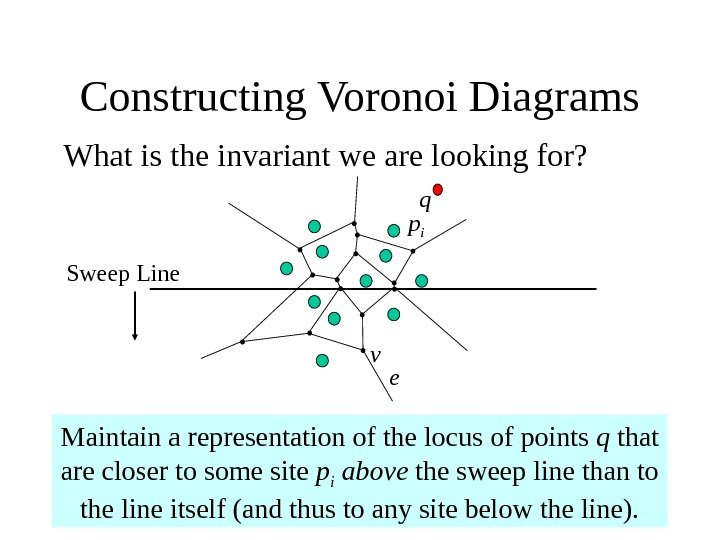Constructing Voronoi Diagrams What is the invariant we are looking for? Maintain a representation of the locus of points q that are closer to some site p i above the sweep line than to the line itself (and thus to any site below the line). ev p i Sweep Line q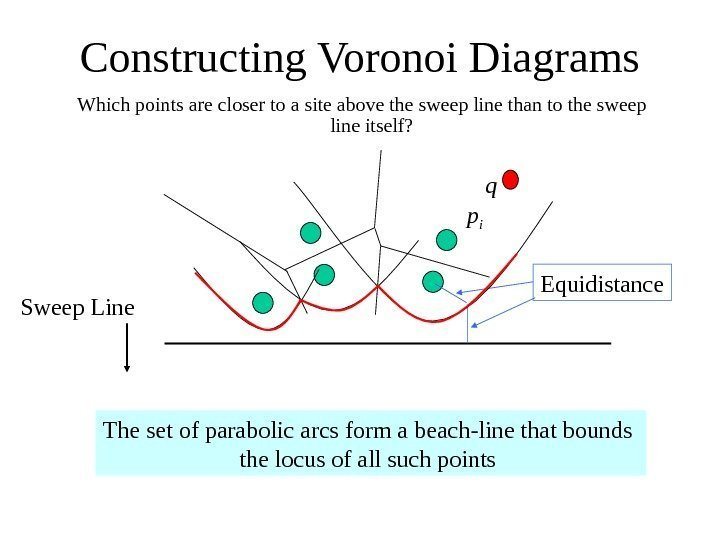Constructing Voronoi Diagrams Which points are closer to a site above the sweep line than to the sweep line itself? Sweep Line p i q The set of parabolic arcs form a beach-line that bounds the locus of all such points Equidistance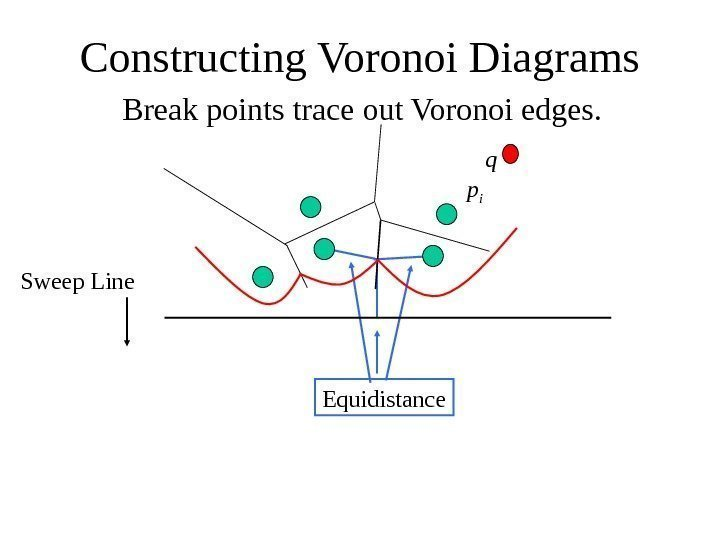Constructing Voronoi Diagrams Break points trace out Voronoi edges. Equidistance. Sweep Line p i q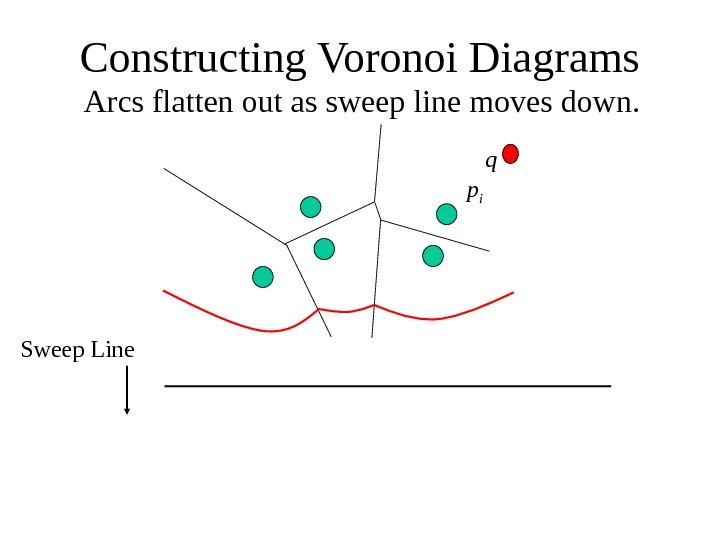Constructing Voronoi Diagrams Arcs flatten out as sweep line moves down. Sweep Line p i q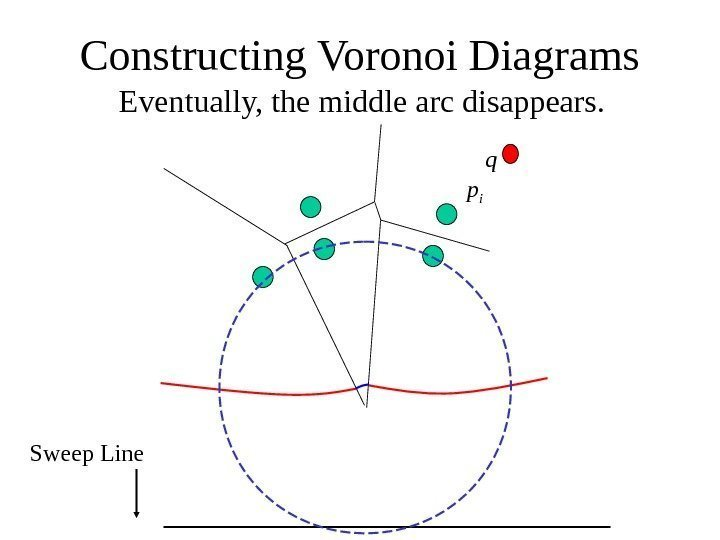Eventually, the middle arc disappears. Constructing Voronoi Diagrams Sweep Line p i q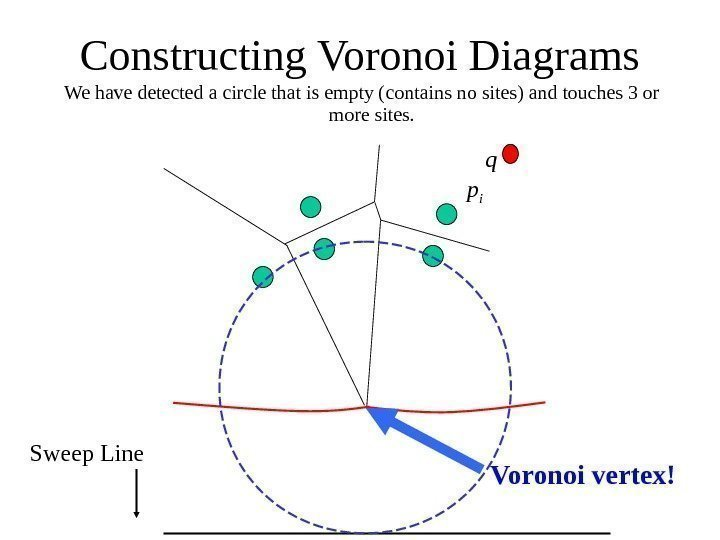We have detected a circle that is empty (contains no sites) and touches 3 or more sites. Constructing Voronoi Diagrams Sweep Line p i q Voronoi vertex!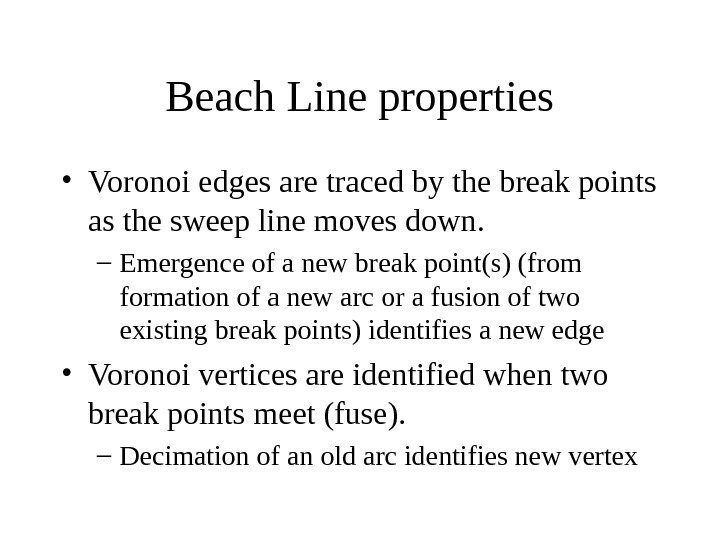Beach Line properties • Voronoi edges are traced by the break points as the sweep line moves down. – Emergence of a new break point(s) (from formation of a new arc or a fusion of two existing break points) identifies a new edge • Voronoi vertices are identified when two break points meet (fuse). – Decimation of an old arc identifies new vertex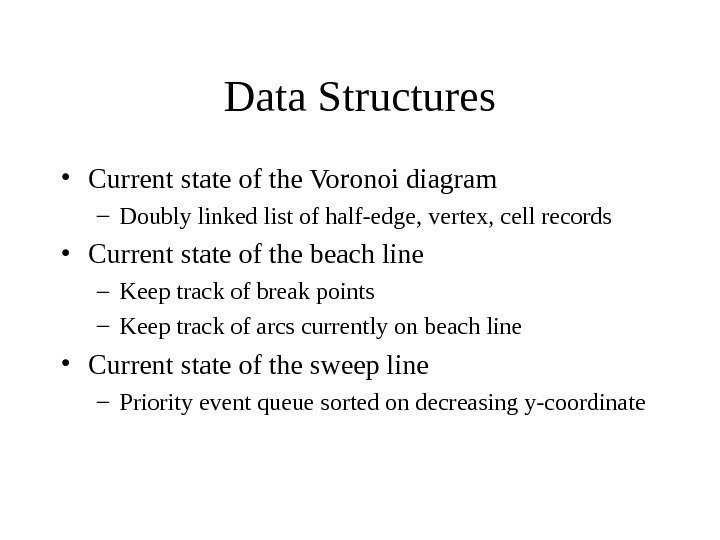Data Structures • Current state of the Voronoi diagram – Doubly linked list of half-edge, vertex, cell records • Current state of the beach line – Keep track of break points – Keep track of arcs currently on beach line • Current state of the sweep line – Priority event queue sorted on decreasing y-coordinate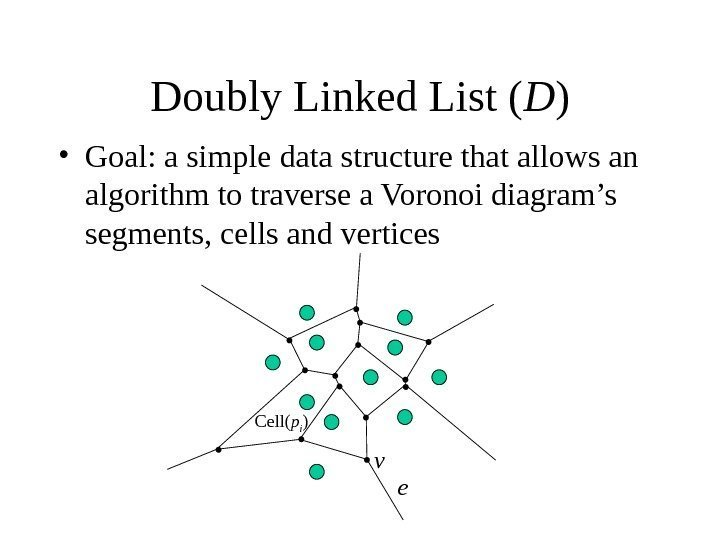Doubly Linked List ( D ) • Goal: a simple data structure that allows an algorithm to traverse a Voronoi diagram’s segments, cells and vertices ev. Cell( p i )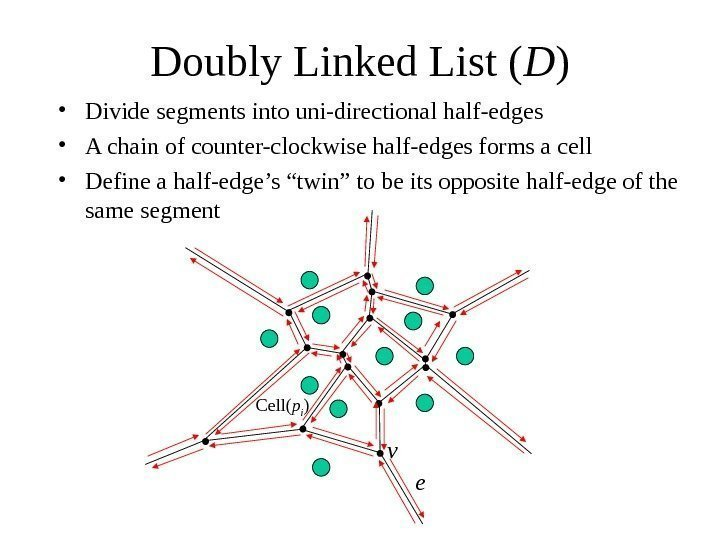Doubly Linked List ( D ) • Divide segments into uni-directional half-edges • A chain of counter-clockwise half-edges forms a cell • Define a half-edge’s “twin” to be its opposite half-edge of the same segment ev Cell( p i )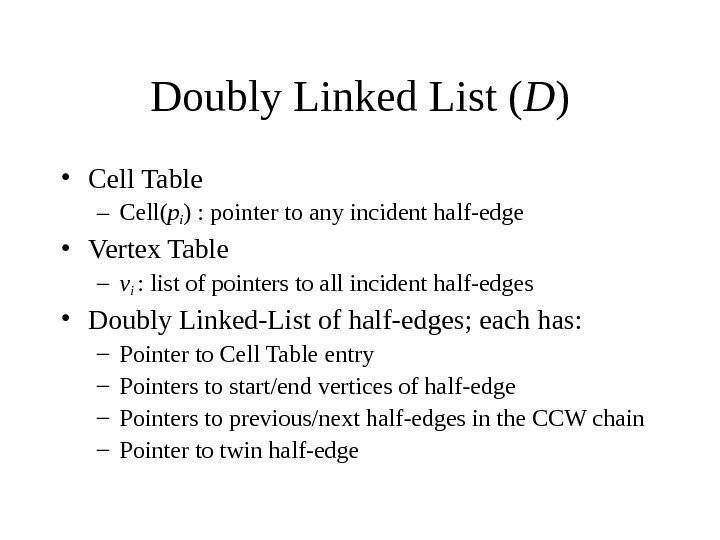Doubly Linked List ( D ) • Cell Table – Cell( pi ) : pointer to any incident half-edge • Vertex Table – v i : list of pointers to all incident half-edges • Doubly Linked-List of half-edges; each has: – Pointer to Cell Table entry – Pointers to start/end vertices of half-edge – Pointers to previous/next half-edges in the CCW chain – Pointer to twin half-edge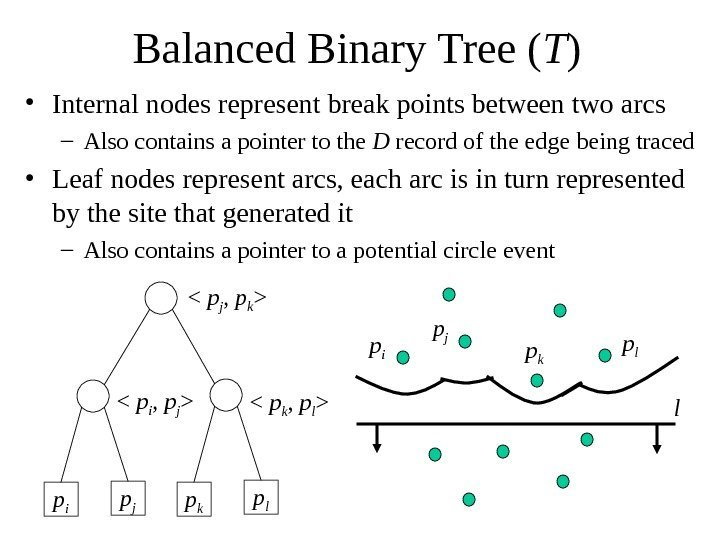Balanced Binary Tree ( T ) • Internal nodes represent break points between two arcs – Also contains a pointer to the D record of the edge being traced • Leaf nodes represent arcs, each arc is in turn represented by the site that generated it – Also contains a pointer to a potential circle event p i p j p k p l

p i p j p k p l l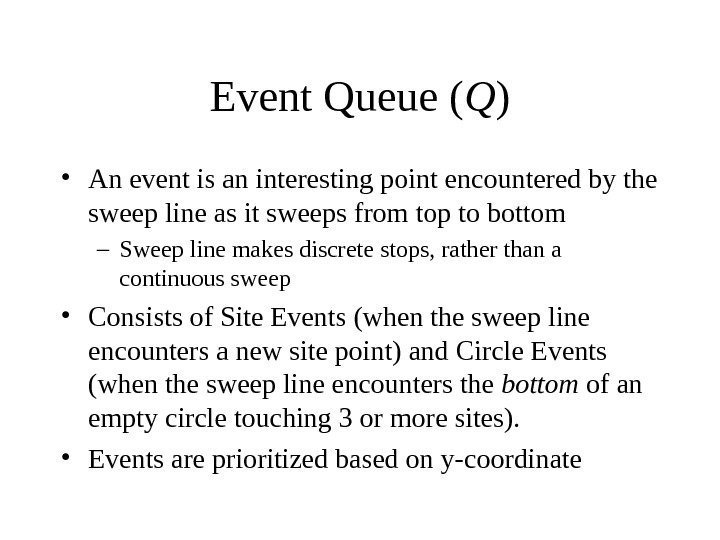Event Queue ( Q ) • An event is an interesting point encountered by the sweep line as it sweeps from top to bottom – Sweep line makes discrete stops, rather than a continuous sweep • Consists of Site Events (when the sweep line encounters a new site point) and Circle Events (when the sweep line encounters the bottom of an empty circle touching 3 or more sites). • Events are prioritized based on y-coordinate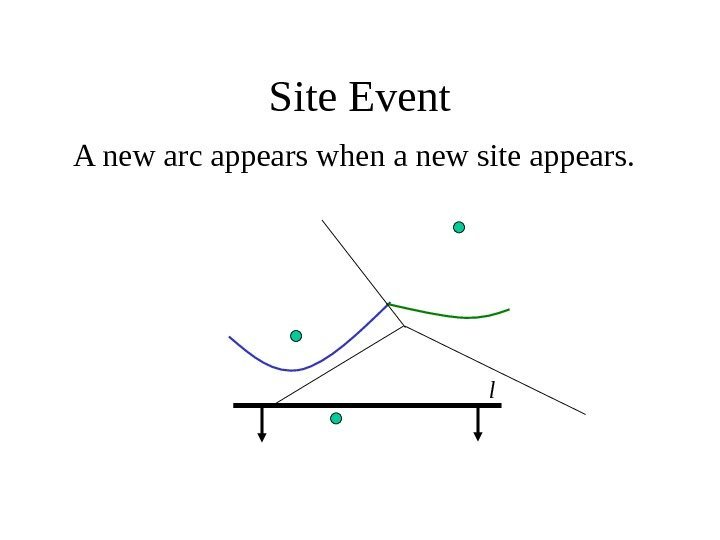Site Event A new arc appears when a new site appears. l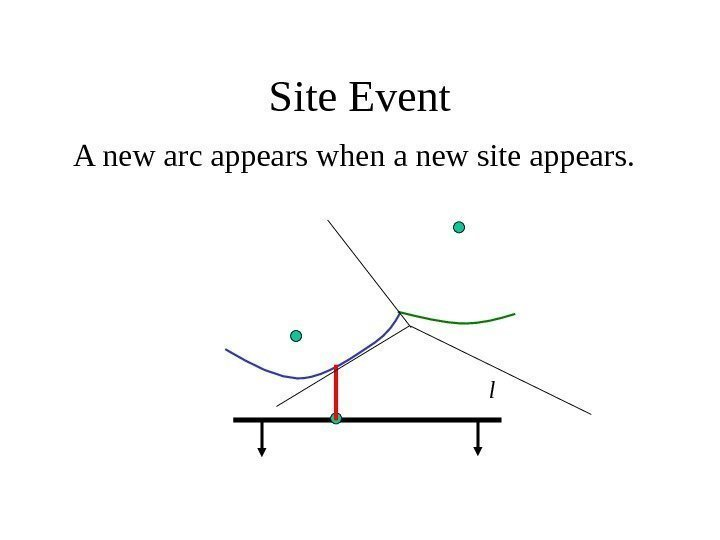Site Event A new arc appears when a new site appears. l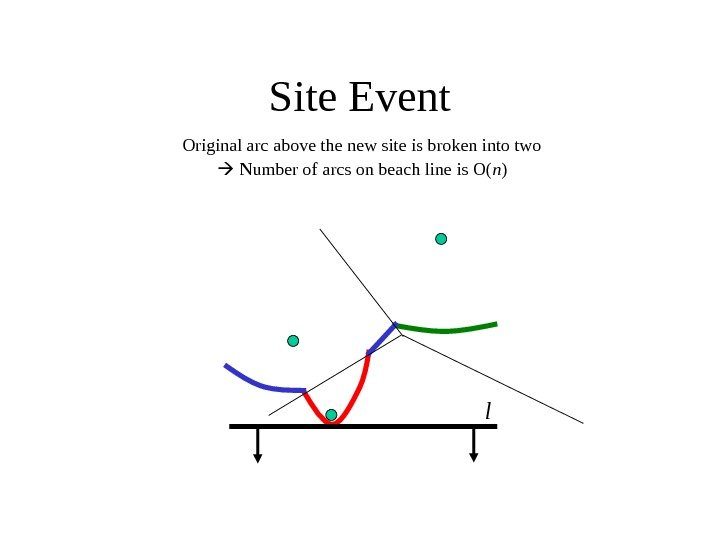Site Event Original arc above the new site is broken into two Number of arcs on beach line is O( n ) l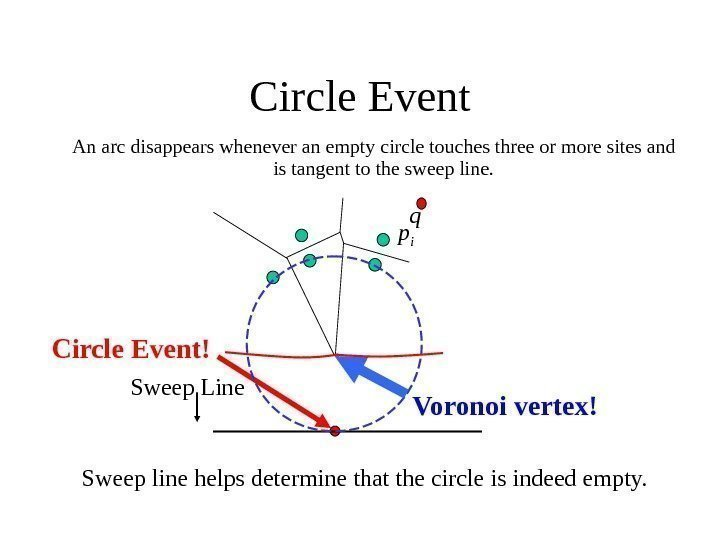Circle Event An arc disappears whenever an empty circle touches three or more sites and is tangent to the sweep line. Sweep line helps determine that the circle is indeed empty. Circle Event! Sweep Line p iq Voronoi vertex!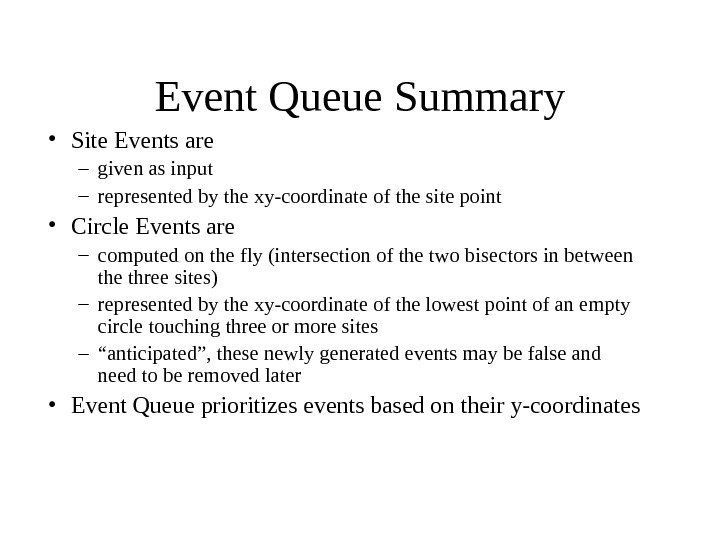Event Queue Summary • Site Events are – given as input – represented by the xy-coordinate of the site point • Circle Events are – computed on the fly (intersection of the two bisectors in between the three sites) – represented by the xy-coordinate of the lowest point of an empty circle touching three or more sites – “ anticipated”, these newly generated events may be false and need to be removed later • Event Queue prioritizes events based on their y-coordinates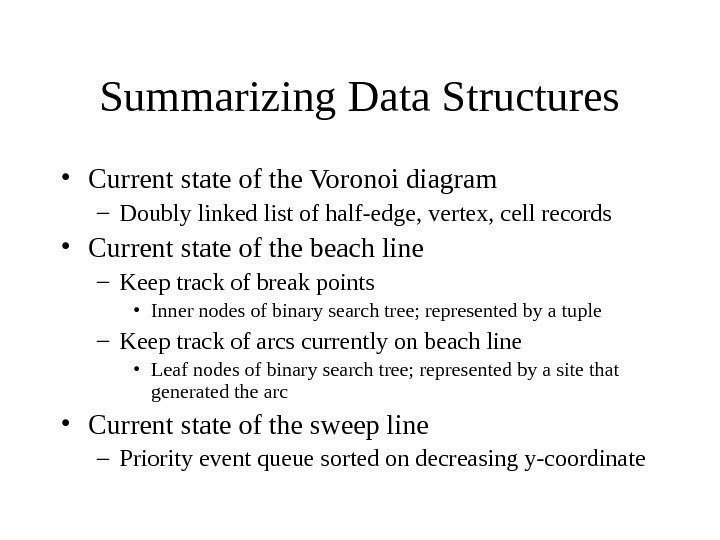Summarizing Data Structures • Current state of the Voronoi diagram – Doubly linked list of half-edge, vertex, cell records • Current state of the beach line – Keep track of break points • Inner nodes of binary search tree; represented by a tuple – Keep track of arcs currently on beach line • Leaf nodes of binary search tree; represented by a site that generated the arc • Current state of the sweep line – Priority event queue sorted on decreasing y-coordinate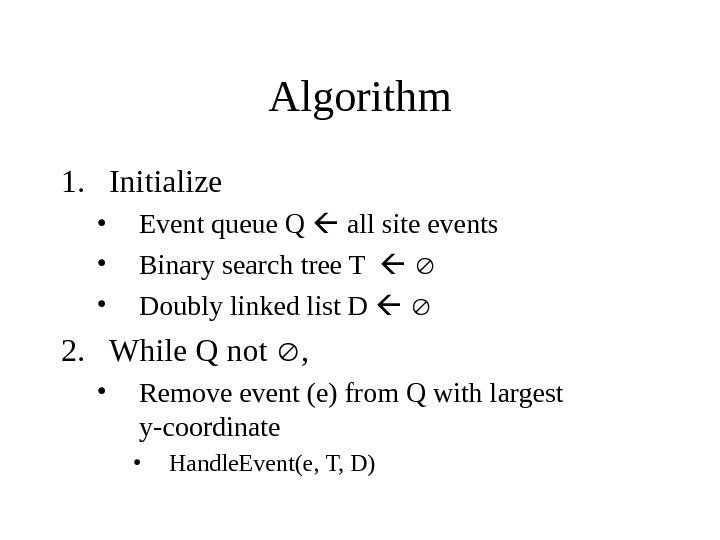Algorithm 1. Initialize • Event queue Q all site events • Binary search tree T • Doubly linked list D 2. While Q not , • Remove event (e) from Q with largest y-coordinate • Handle. Event(e, T, D)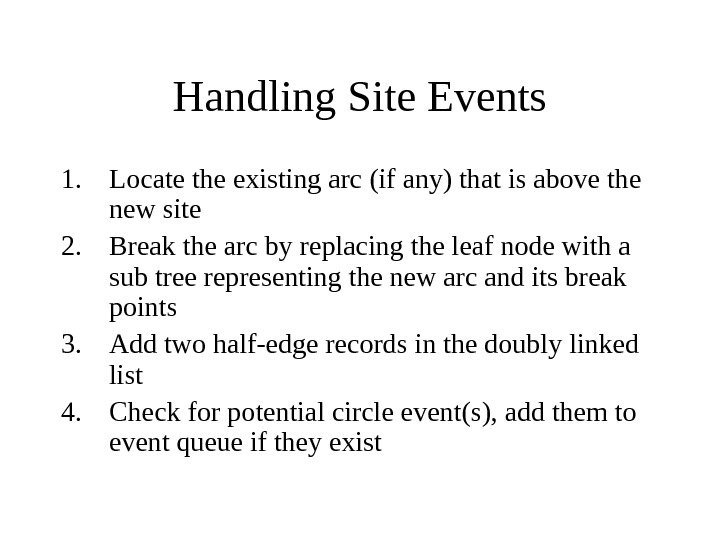Handling Site Events 1. Locate the existing arc (if any) that is above the new site 2. Break the arc by replacing the leaf node with a sub tree representing the new arc and its break points 3. Add two half-edge records in the doubly linked list 4. Check for potential circle event(s), add them to event queue if they exist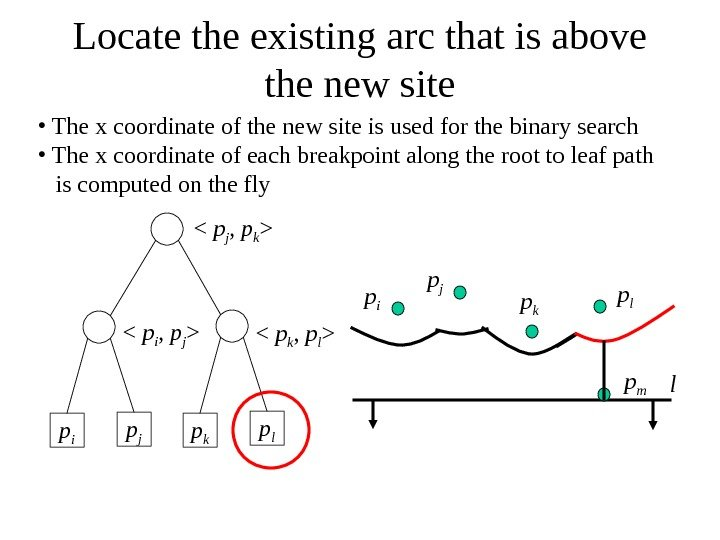Locate the existing arc that is above the new site p i p j p k p l

• The x coordinate of the new site is used for the binary search • The x coordinate of each breakpoint along the root to leaf path is computed on the fly p i p j p k p l lp m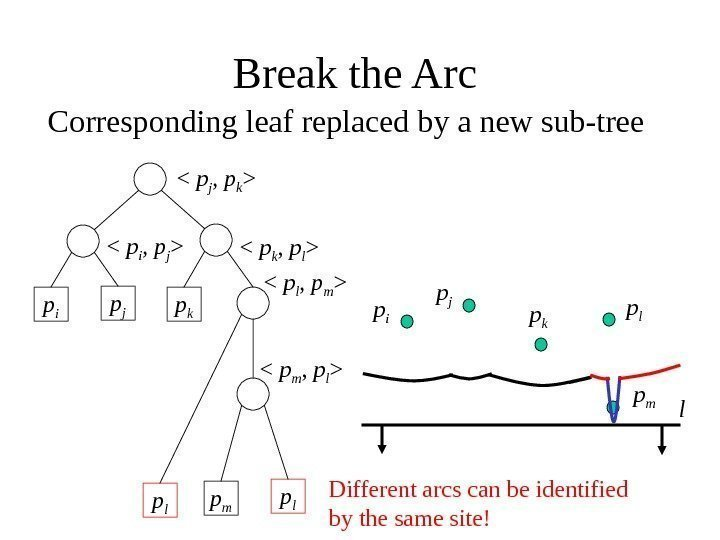Break the Arc p i p j p k

Corresponding leaf replaced by a new sub-tree p i p j p k p l lp m p l

p l Different arcs can be identified by the same site!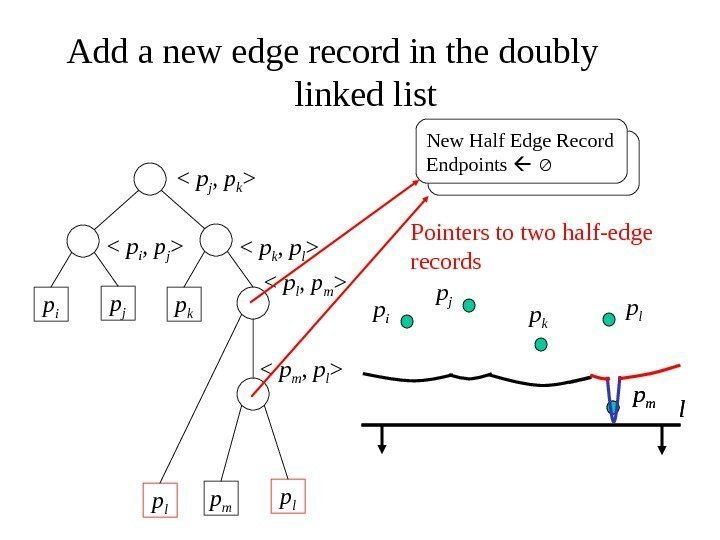Add a new edge record in the doubly linked list p i p j p k

p m p l

p l p i p j p k p l lp m. New Half Edge Record Endpoints Pointers to two half-edge records lp m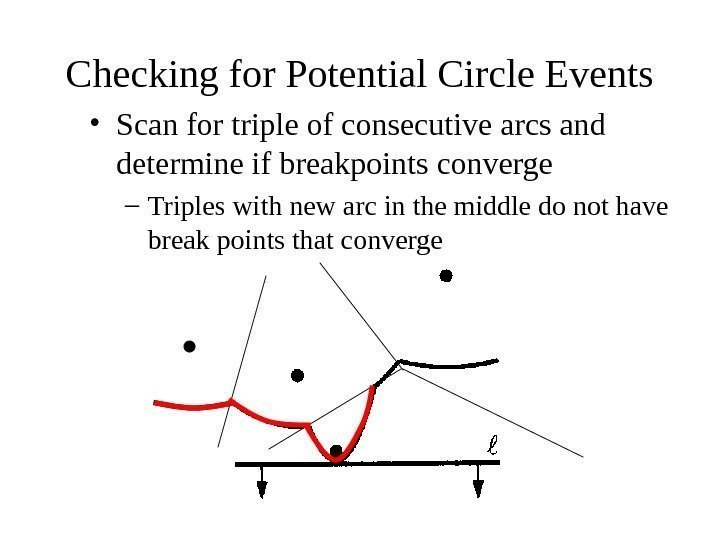Checking for Potential Circle Events • Scan for triple of consecutive arcs and determine if breakpoints converge – Triples with new arc in the middle do not have break points that converge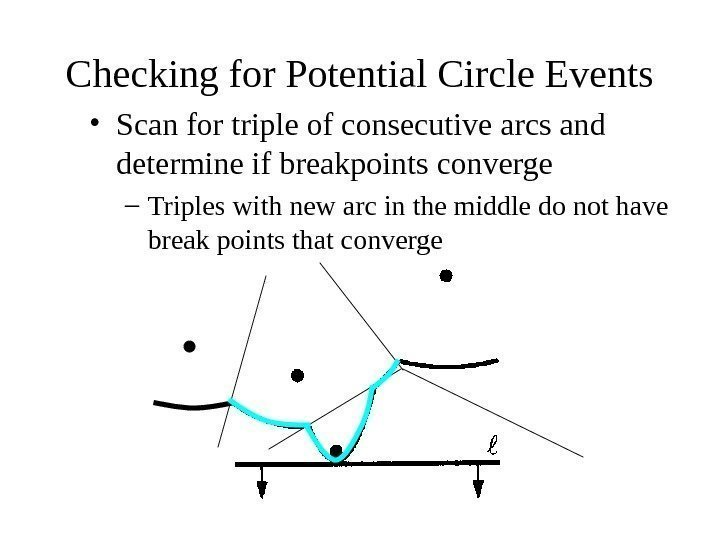Checking for Potential Circle Events • Scan for triple of consecutive arcs and determine if breakpoints converge – Triples with new arc in the middle do not have break points that converge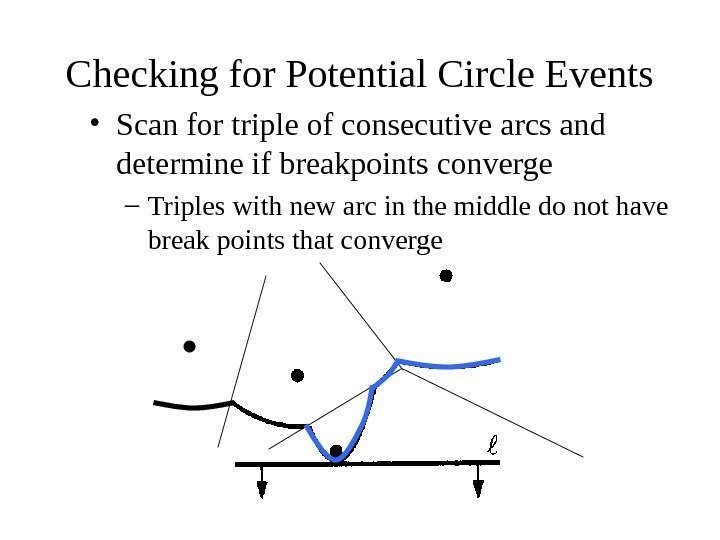Checking for Potential Circle Events • Scan for triple of consecutive arcs and determine if breakpoints converge – Triples with new arc in the middle do not have break points that converge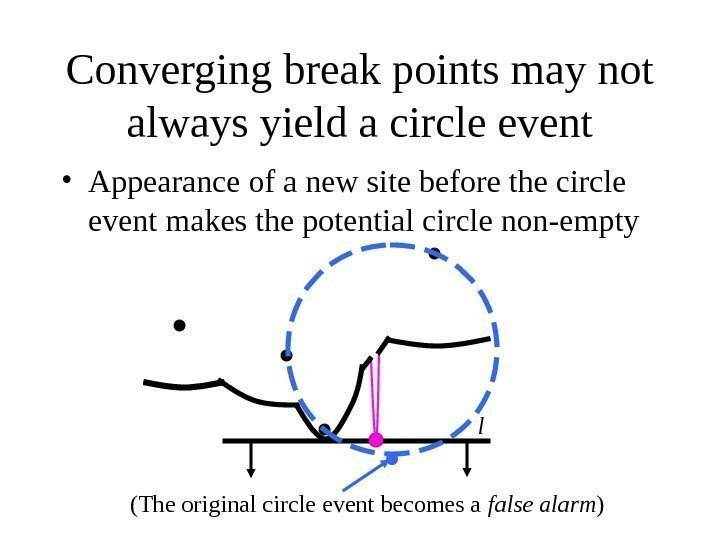Converging break points may not always yield a circle event • Appearance of a new site before the circle event makes the potential circle non-empty l (The original circle event becomes a false alarm )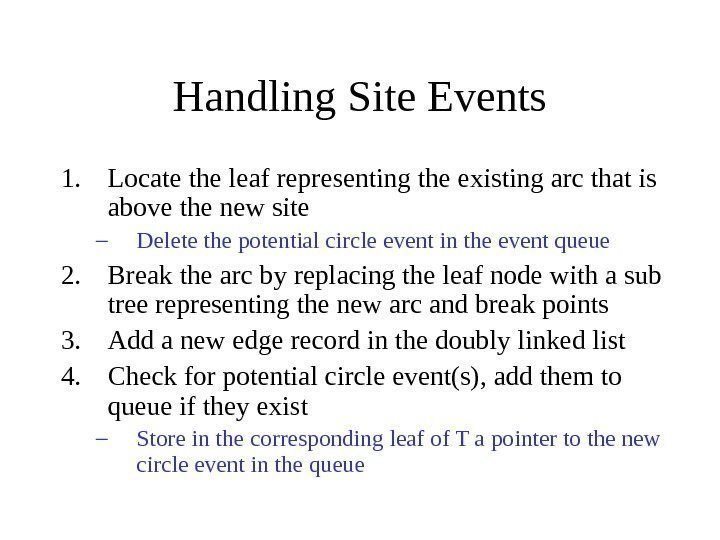Handling Site Events 1. Locate the leaf representing the existing arc that is above the new site – Delete the potential circle event in the event queue 2. Break the arc by replacing the leaf node with a sub tree representing the new arc and break points 3. Add a new edge record in the doubly linked list 4. Check for potential circle event(s), add them to queue if they exist – Store in the corresponding leaf of T a pointer to the new circle event in the queue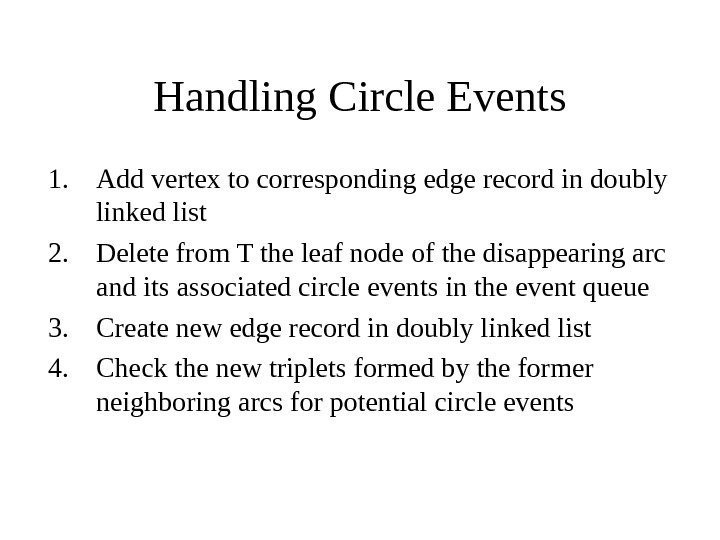Handling Circle Events 1. Add vertex to corresponding edge record in doubly linked list 2. Delete from T the leaf node of the disappearing arc and its associated circle events in the event queue 3. Create new edge record in doubly linked list 4. Check the new triplets formed by the former neighboring arcs for potential circle events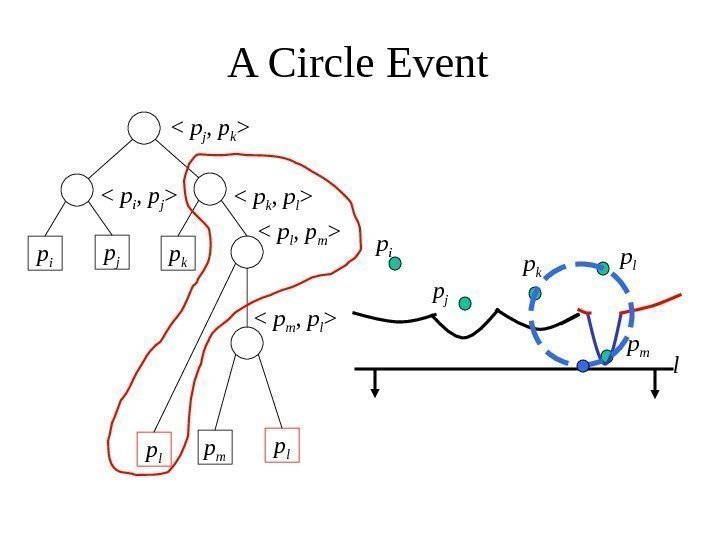A Circle Event p i p j p k

p i p j p k p l lp m p l

p l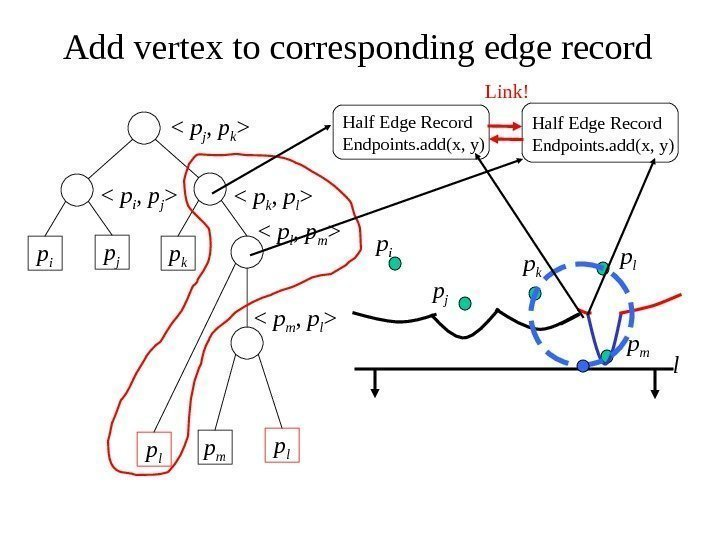Add vertex to corresponding edge record p i p j p k

p i p j p k p l lp m p l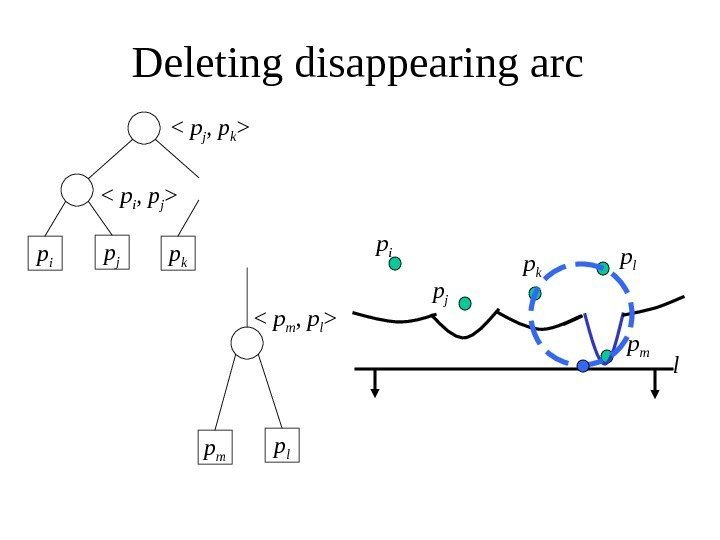Deleting disappearing arc p i p j p k

p i p j p k p l lp m p l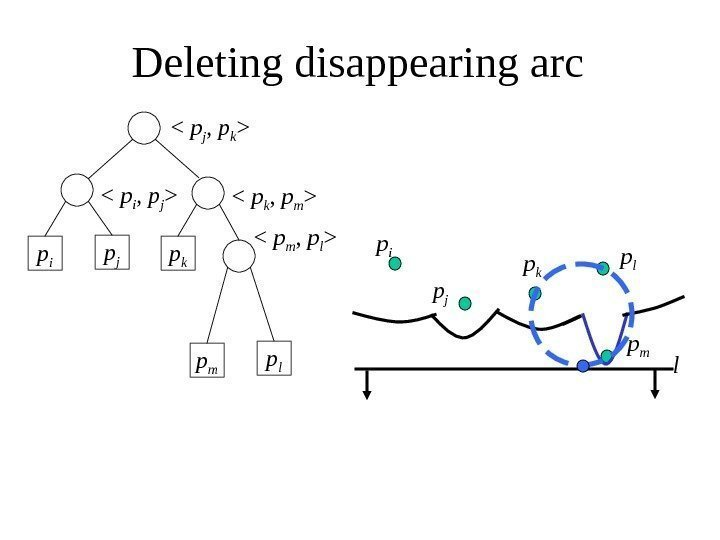Deleting disappearing arc p i p j p k

p i p j p k p l lp m p l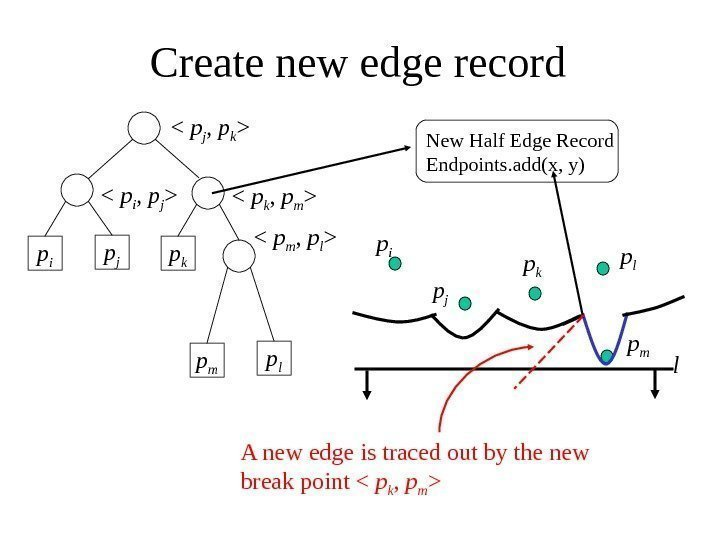Create new edge record p i p j p k

p i p j p k p l lp m p l

New Half Edge Record Endpoints. add(x, y) A new edge is traced out by the new break point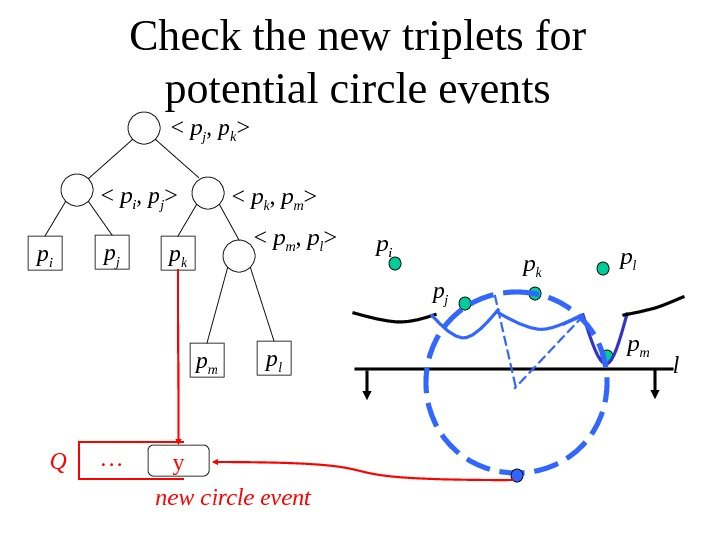Check the new triplets for potential circle events p i p j p k

p i p j p k p l lp m p l

Q y… new circle event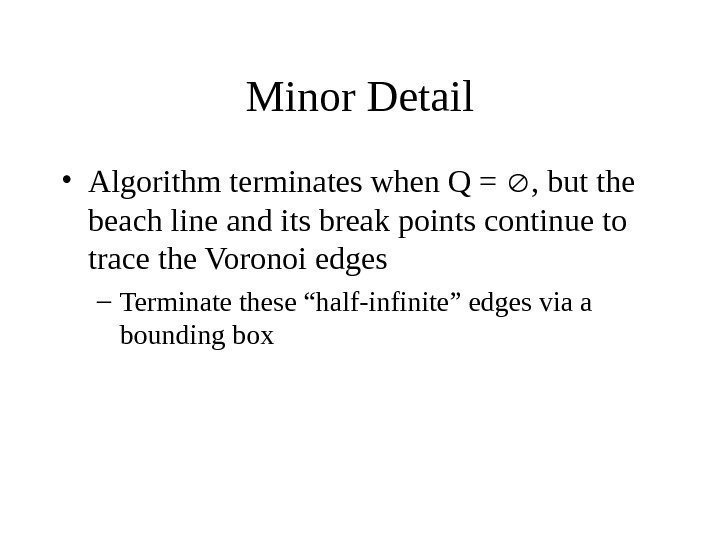Minor Detail • Algorithm terminates when Q = , but the beach line and its break points continue to trace the Voronoi edges – Terminate these “half-infinite” edges via a bounding box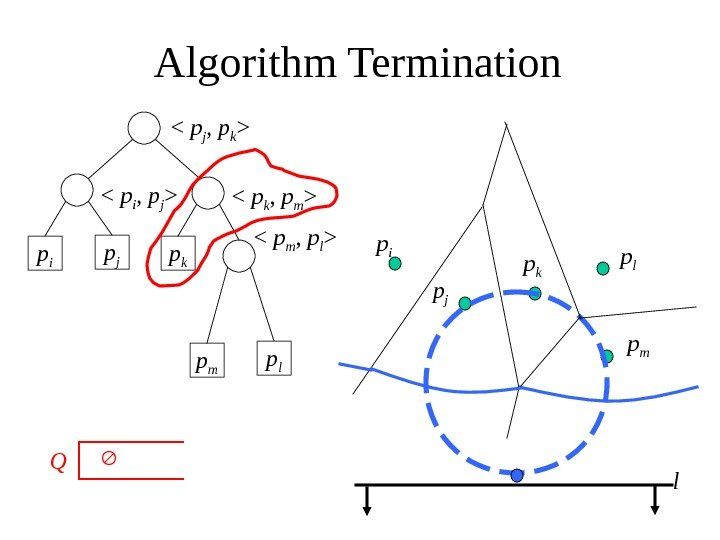Algorithm Termination p i p j p k

p i p j p k p l lp m p l

Q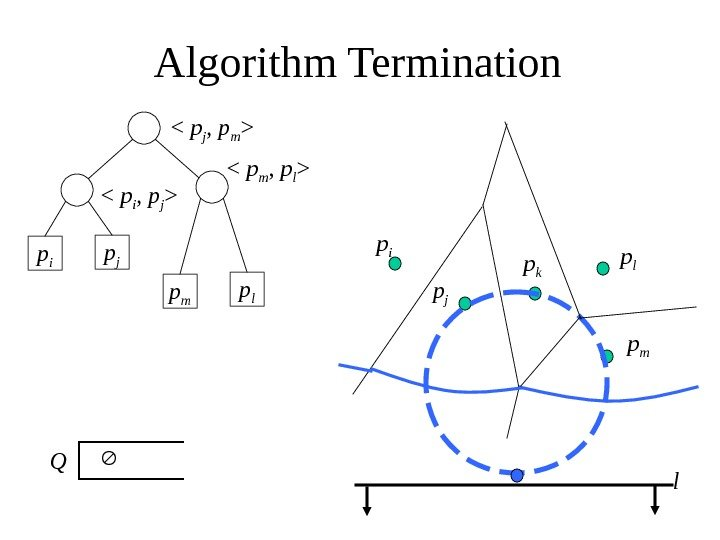Algorithm Termination p i p j

p i p j p k p l lp mp m p l

Q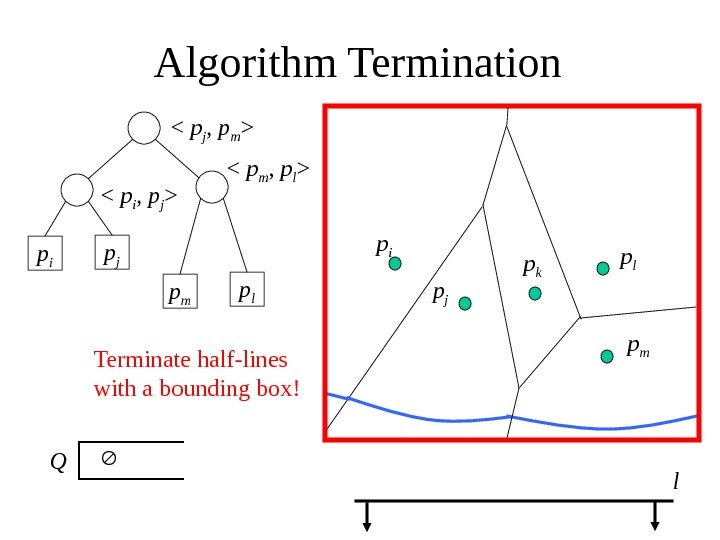Algorithm Termination p i p j

p i p j p k p l lp mp m p l

Q Terminate half-lines with a bounding box!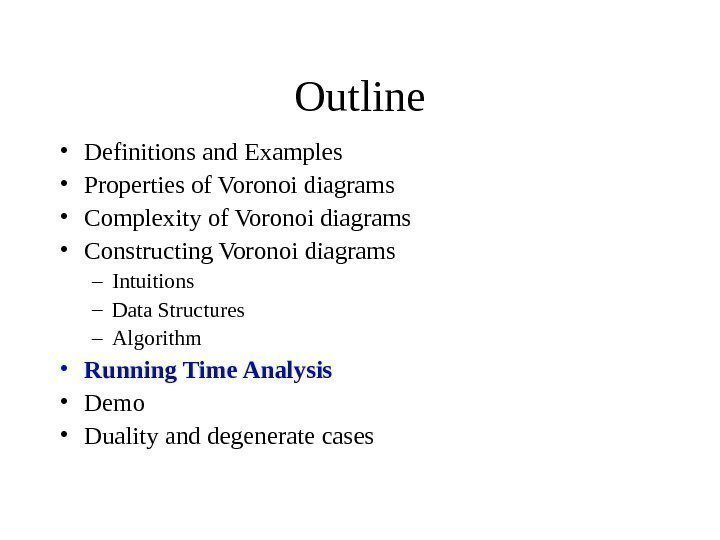Outline • Definitions and Examples • Properties of Voronoi diagrams • Complexity of Voronoi diagrams • Constructing Voronoi diagrams – Intuitions – Data Structures – Algorithm • Running Time Analysis • Demo • Duality and degenerate cases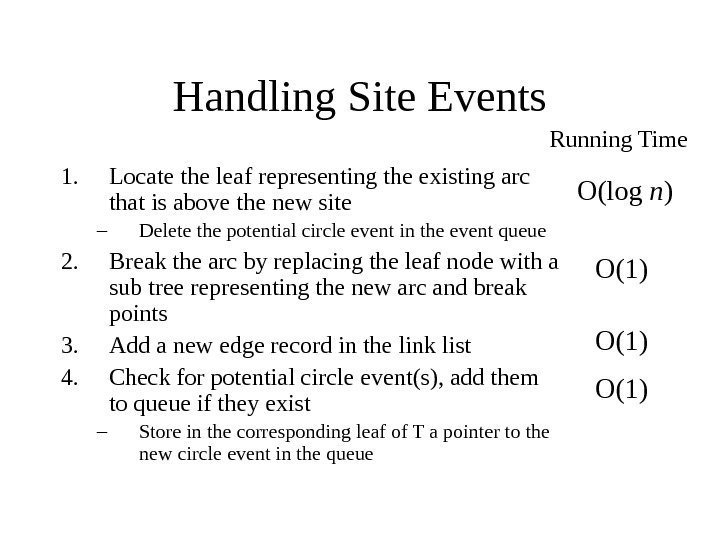Handling Site Events 1. Locate the leaf representing the existing arc that is above the new site – Delete the potential circle event in the event queue 2. Break the arc by replacing the leaf node with a sub tree representing the new arc and break points 3. Add a new edge record in the link list 4. Check for potential circle event(s), add them to queue if they exist – Store in the corresponding leaf of T a pointer to the new circle event in the queue Running Time O(log n ) O(1)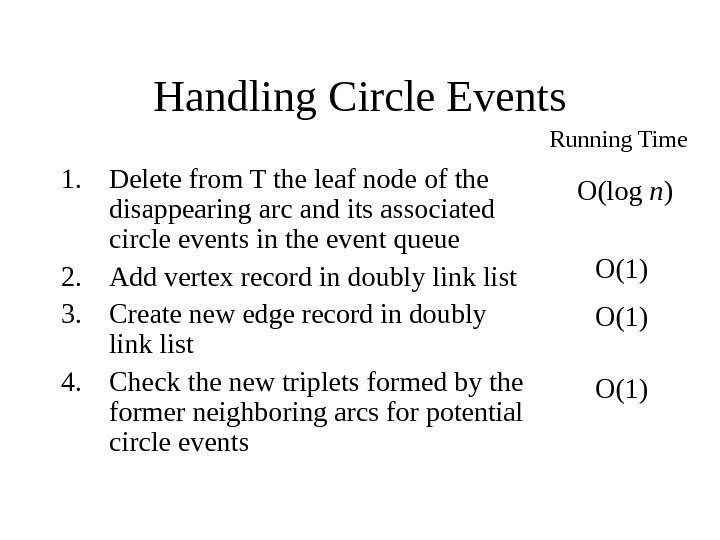Handling Circle Events 1. Delete from T the leaf node of the disappearing arc and its associated circle events in the event queue 2. Add vertex record in doubly link list 3. Create new edge record in doubly link list 4. Check the new triplets formed by the former neighboring arcs for potential circle events Running Time O(log n ) O(1)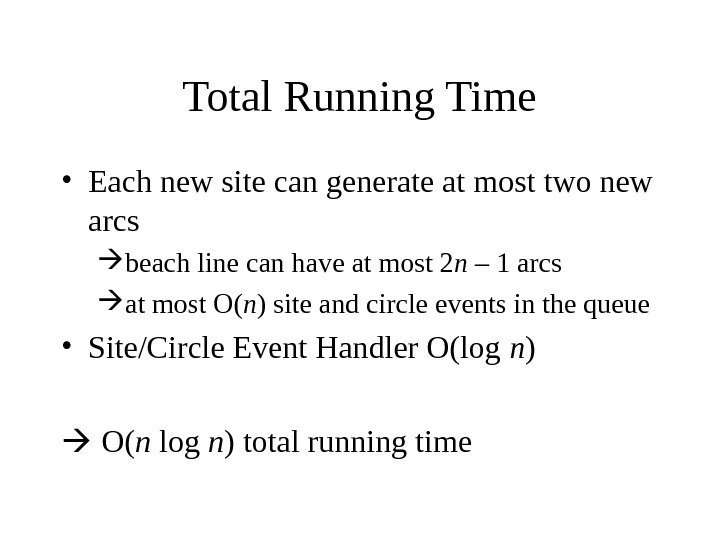Total Running Time • Each new site can generate at most two new arcs beach line can have at most 2 n – 1 arcs at most O( n ) site and circle events in the queue • Site/Circle Event Handler O(log n ) O( n log n ) total running time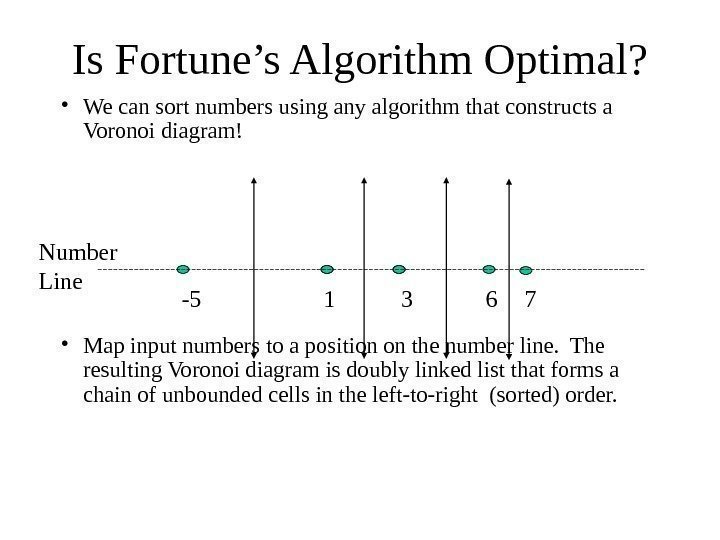Is Fortune’s Algorithm Optimal? • We can sort numbers using any algorithm that constructs a Voronoi diagram! • Map input numbers to a position on the number line. The resulting Voronoi diagram is doubly linked list that forms a chain of unbounded cells in the left-to-right (sorted) order. -5 1 3 6 7 Number Line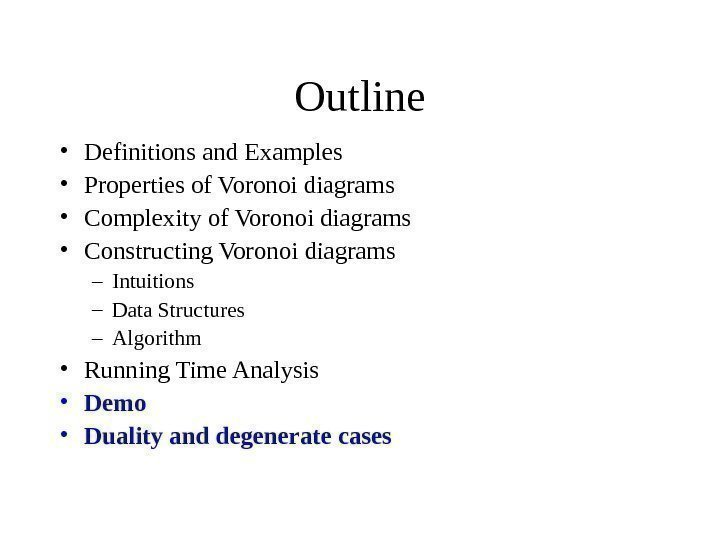Outline • Definitions and Examples • Properties of Voronoi diagrams • Complexity of Voronoi diagrams • Constructing Voronoi diagrams – Intuitions – Data Structures – Algorithm • Running Time Analysis • Demo • Duality and degenerate cases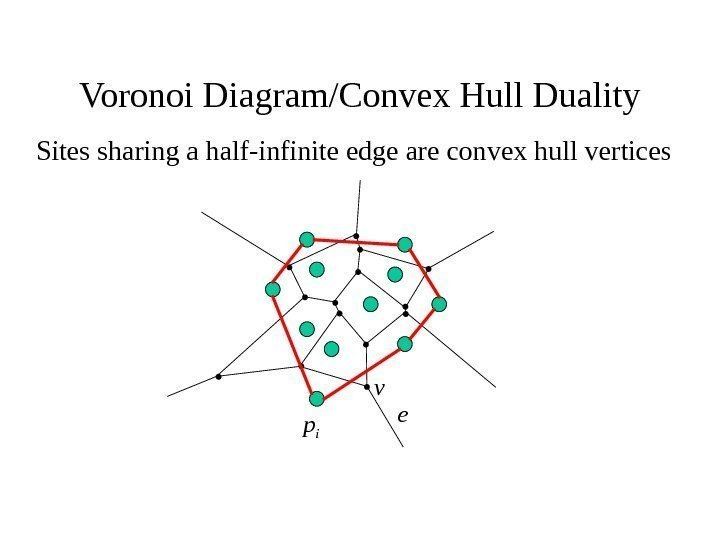Voronoi Diagram/Convex Hull Duality Sites sharing a half-infinite edge are convex hull vertices ev p i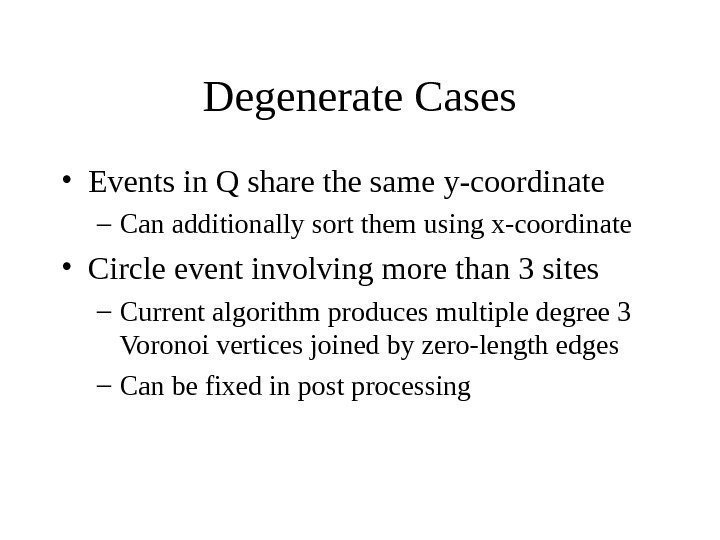Degenerate Cases • Events in Q share the same y-coordinate – Can additionally sort them using x-coordinate • Circle event involving more than 3 sites – Current algorithm produces multiple degree 3 Voronoi vertices joined by zero-length edges – Can be fixed in post processing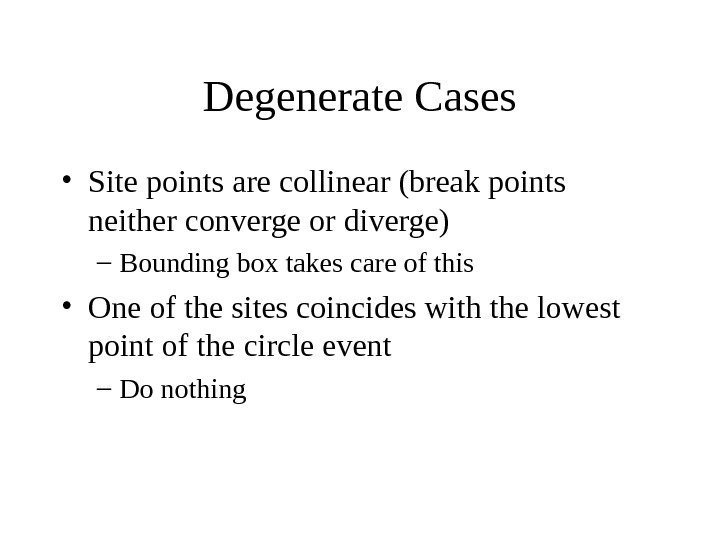Degenerate Cases • Site points are collinear (break points neither converge or diverge) – Bounding box takes care of this • One of the sites coincides with the lowest point of the circle event – Do nothing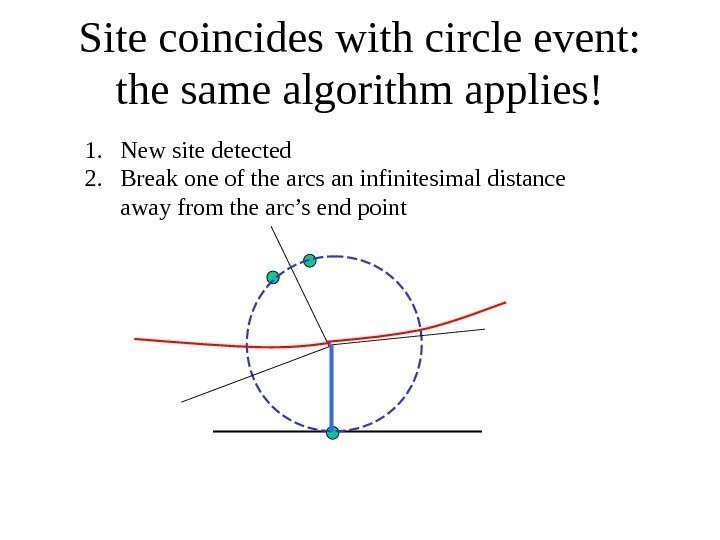Site coincides with circle event: the same algorithm applies! 1. New site detected 2. Break one of the arcs an infinitesimal distance away from the arc’s end point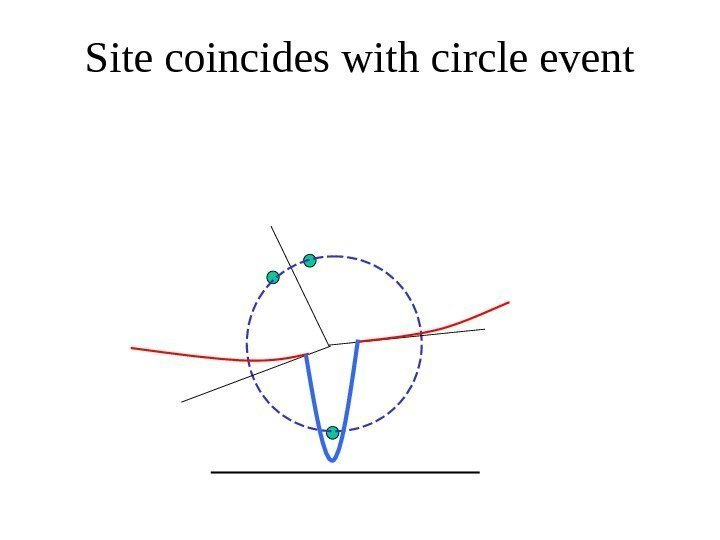Site coincides with circle event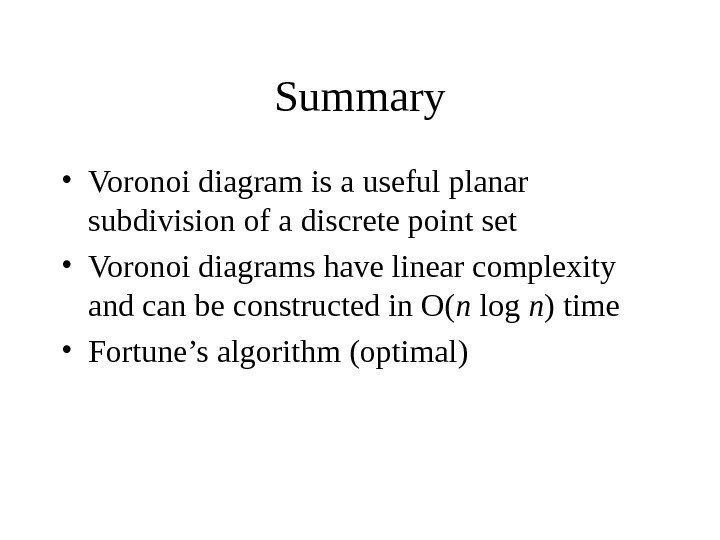Summary • Voronoi diagram is a useful planar subdivision of a discrete point set • Voronoi diagrams have linear complexity and can be constructed in O( n log n ) time • Fortune’s algorithm (optimal)